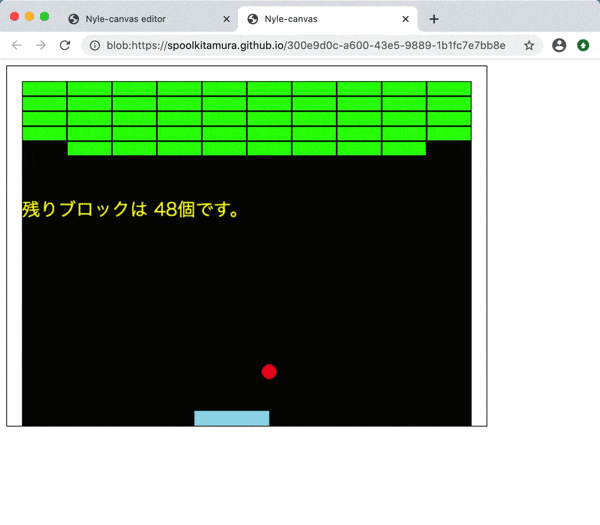# Overview

We will create a "breakout" game using Nyle-canvas, which allows you to start Ruby game programming with just a browser. We will make "breakout" little by little from 0. Even if it is completed with Ruby, "breakout" can be done in more than 100 lines.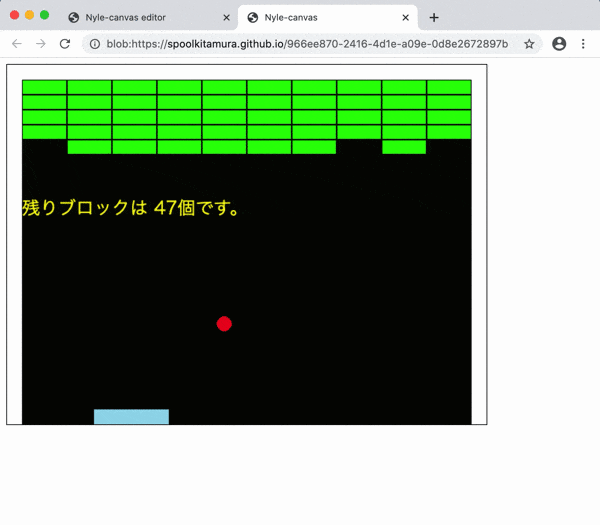# Technical explanation

## Library used

--Nyle-canvas (DXRuby style)

https://spoolkitamura.github.io/nyle-canvas/

Since it is an integrated development environment that integrates a Ruby editor and execution environment that runs on a browser, there is no need to install Ruby.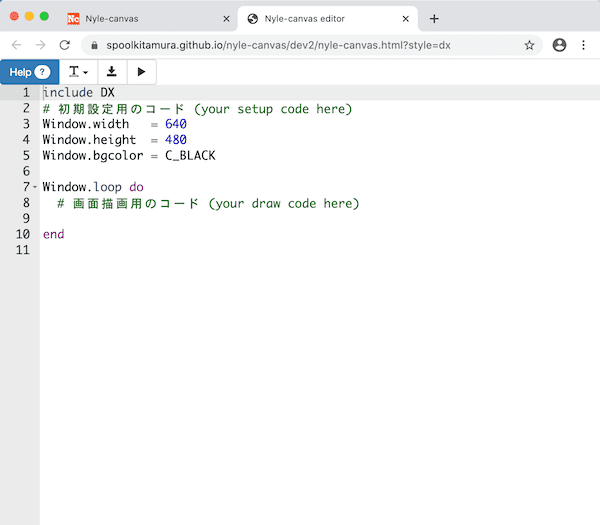## Nyle-canvas (DXRuby style)

In this text, we will use the DXRuby API compatible "DXRuby style" among the Nyle-canvas description styles.

## How to use Nyle-canvas

See the post below.

--Starting Ruby game programming with a browser: Introduction to Nyle-canvas (DXRuby style) --Qiita

https://qiita.com/noanoa07/items/e7ebf97edef4ae9b448a

## Operating environment required for Nyle-canvas

--Browser (Chrome, etc.)

• Internet connection --OS that runs the browser (Windows, macOS, Linux, Android, iPadOS, iOS, etc.) --PC, tablet (with external keyboard), smartphone (* Some touch panel operations are also supported)

## Nyle-canvas homepage

--Nyle-canvas homepage

https://spoolkitamura.github.io/nyle-canvas/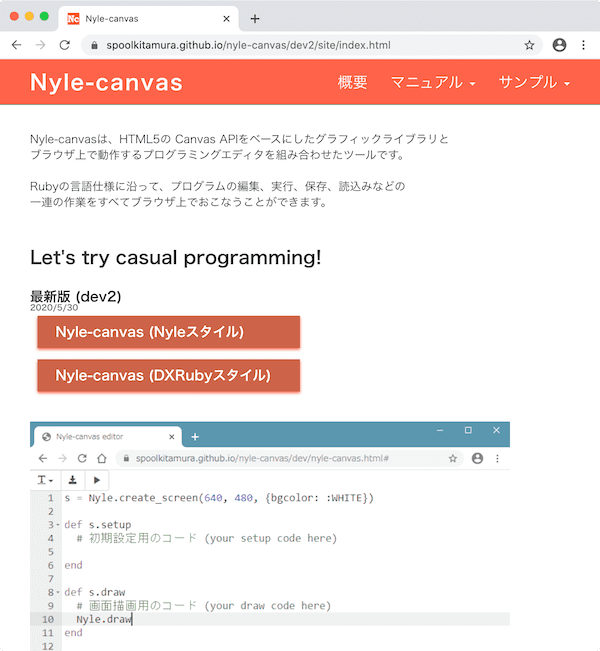--Nyle-canvas (DXRuby style) editor; editor screen for actual programming

From "Nyle-canvas homepage", click the "DXRuby style" link.--Nyle-canvas manual; how to operate the editor

https://spoolkitamura.github.io/nyle-canvas/dev2/site/manual_editor.html

## Reference site

DXRuby page which is the original of "DXRuby style";

--DXRuby homepage http://dxruby.osdn.jp

--DXRuby 1.4.6 Reference Manual http://mirichi.github.io/dxruby-doc/index.html

--DXRuby 1.4.1 Reference Manual http://dxruby.osdn.jp/DXRubyReference/20095313382446.htm

An article explaining how to use DXRuby;

--2D game library for Ruby DXRuby: Basics of how to use --Qiita https://qiita.com/noanoa07/items/bced6519d9b53685b651

Other DXRuby API compatible libraries that work in the browser;

• DXOpal https://yhara.github.io/dxopal/doc/ja/index.html

https://yhara.github.io/dxopal/index.html

--Game programming starting with Ruby --DXOpal edition --Rubyist Magazine No. 0057 https://magazine.rubyist.net/articles/0057/0057-GameProgramingWithDXOpal.html

The article "Breakout" made with DXRuby, which is the basis of this text;

--For programming beginners: "Breakout" that creates step by step with DXRuby --Qiita https://qiita.com/noanoa07/items/9ebc059550c620ab223c

## Execution environment of this text

--Browser; Google Chrome (version 83.0.4103.61, 64-bit, macOS version)

--Execution OS; macOS（Catalina 10.15.5）

In addition, we have confirmed the operation on Safari / macOS and Chrome / Windows10 as appropriate.

## Corresponding version

Nyle-canvas (DXRuby style); dev2 (released 5/30/2020)

## original

• Modified from "2014-03-17 I participated in Matsue Ruby Conference 05 --mirichi's diary"

http://d.hatena.ne.jp/mirichi/20140317/p1

## Source code of this text

https://github.com/noanoa07/nyle-canvas-blocks

The commentary, Ruby source code, and image data in this text are all in the public domain.

(Nyle-canvas source code is MIT licensed)

# Program explanation

## 1. 1. How to use Nyle-canvas (outline)

See the post below for how to use Nyle-canvas.

--Starting Ruby game programming with a browser: Introduction to Nyle-canvas (DXRuby style) --Qiita https://qiita.com/noanoa07/items/e7ebf97edef4ae9b448a

Here is the minimum explanation.

### 1-1. Open Nyle-canvas editor

For Nyle-canvas, the program edit screen (editor) is displayed by accessing the following site with a browser.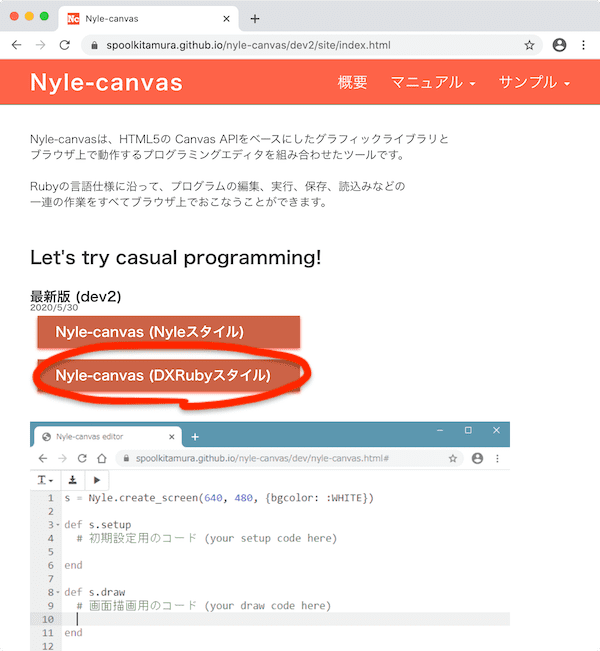"Nyle-canvas homepage" https://spoolkitamura.github.io/nyle-canvas/ From Click the "DX Ruby Style" link.This is the Nyle-canvas editor screen.

In addition, here we will explain using Google Chrome as the browser. (The execution environment is macOS.)

The basic program is displayed in advance on the editor screen. In addition, various buttons are lined up in the upper left of the screen. From the right --`▶ ︎ button` ； Program execution --`↓ button`; Save program (download) --`T button`; Font (character size) setting --`? Button`; Go to help (reference) screen

#### ・ Create a new program

--Press the `" ↻ "button` at the top of the browser --Select `Menu`>` File`> `View`> `Reload Page` --Shortcut; (macOS) `Command` +` R`, (Windows) `Ctrl` +` R`

### 1-2. Program execution / re-execution

The program is ** no save operation ** and will be executed ** immediately ** just by pressing the `▶ ︎ button`.

``````include DX
#Code for initial setting(your setup code here)
Window.width   = 640
Window.height  = 480
Window.bgcolor = C_BLACK

Window.loop do
#Code for screen drawing(your draw code here)

end
``````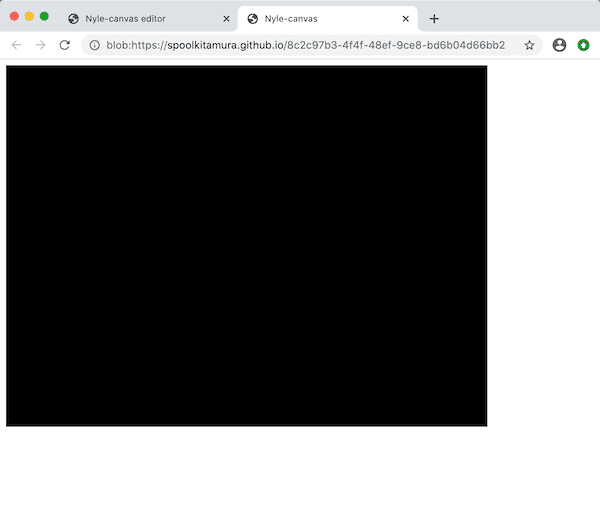A new browser tab opens with a basic black square window.

The coordinates are the origin (x = 0, y = 0) at the upper left, the horizontal (x) increasing to the right, and the vertical (y) increasing downward.

The code written between `Window.loop do ~ end` is executed 60 times a second repeatedly.

→ DXRuby Reference: Tutorial 1. Basic form http://mirichi.github.io/dxruby-doc/tutorial/basic.html

#### ・ Re-execution

You can re-execute the program by doing ** "Reload" on the ** execution screen.

--Press the `" ↻ "button` at the top of the browser --Select `Menu`>` File`> `View`> `Reload Page` --Shortcut; (macOS) `Command` +` R`, (Windows) `Ctrl` +` R`

#### ・ Close the execution screen

Close the tab on the execution screen. Press the `" × "button` at the top of the screen.

--Shortcut; (macOS) `Command` +` W`, (Windows) `Ctrl` +` W`

Press the `↓ button` to download the program. The download destination depends on the browser settings, but it seems that it is often the "Download" folder.

Drag and drop the Nyle-canvas program file (`HTML file`) onto the` Nyle-canvas editor screen` of your browser.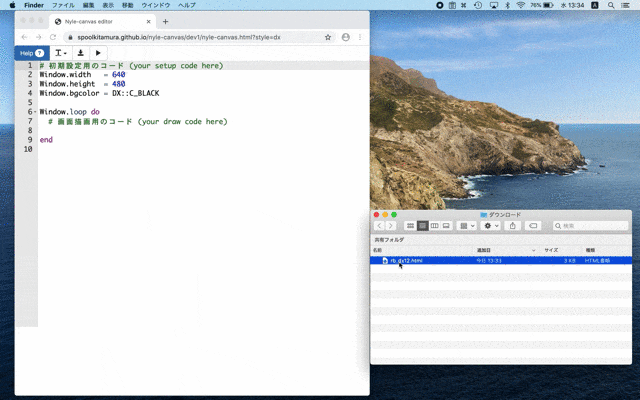Nyle-Canvas also saves and manages image files together with the program.

The image file you want to use can be copied into Nyle-canvas by `drag and drop` directly to the editor screen, and will be displayed as a list of image files at the bottom of the editor screen. (* Uppercase letters may be lowercase.)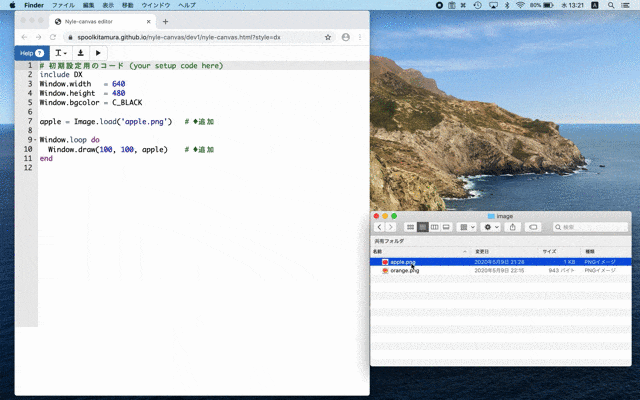To load and use an image file from a program, use ʻImage.load (image file name)`. The ** path name etc. that indicates the location before the `image file name` is unnecessary **.

The loaded image will be in the ʻImage class`.

### 1-6. How to open the console

Results such as `error messages` and` puts` at runtime are printed to the browser's `console`.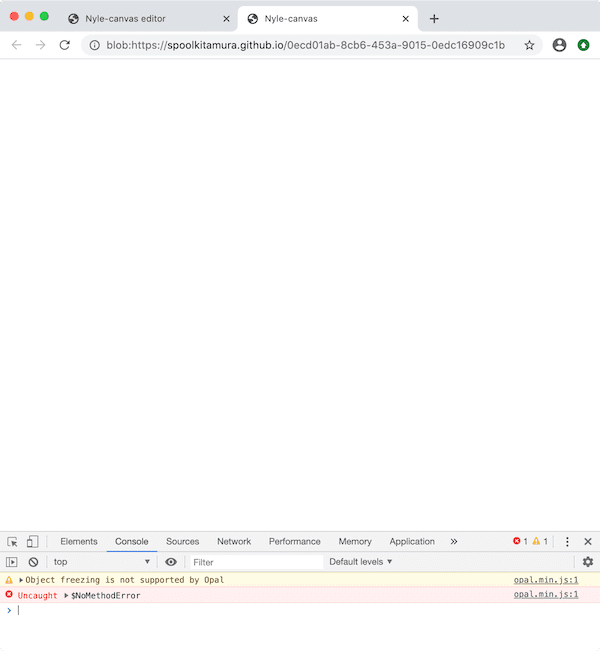How to open in Chrome; (** on the execution screen **)

#### a) Common to macOS and Windows

--Press the `F12` (or` fn` + `F12`) key -(Anywhere on the screen); `Right click` (two-finger click, etc.)>` Verification`> Select `" Console "` in the opened developer tools

b) macOS --Select `Menu`>` Display`> `Development / Management`>` Javascript Console` --Shortcut; `Command` + ʻOption` +` J`

c) Windows --`Menu``Other Tools``Developer Tools` ＞ Select`"Console"`from the opened Developer Tools --Shortcut; `Ctrl` +` Shift` + `J`

### 1-7. Help (reference)

Press the `"? "Button` at the top left of the Nyle-canvas editor screen to open the help (reference) screen.

→ \ [Nyle-canvas] API Reference (DXRuby style) https://spoolkitamura.github.io/nyle-canvas/dev2/site/_man_api_dx/index.html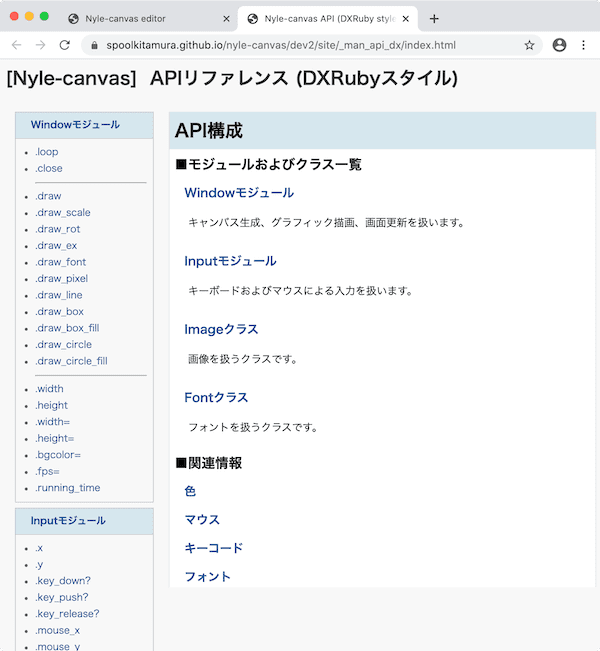The API of Nyle-canvas is summarized briefly, so please use it more and more when you are in trouble.

## 2. Make a "breakout"

Finally, we will make a "breakout".

#### * About `.html file` and` .rb file`

The program that runs on Nyle-canvas is the `.html file`. (The sample program is in the `src / block_html folder`.)

However, in the following explanation, only the program part of Ruby will be listed. (There is a `.rb file` with the same name in the` src / block_ruby folder`.)

### 2-1. Put out the wall (left side) (rb_block01.html)

First, make a vertical wall on the left side (thickness 20).

Make the upper left corner `(0, 0)`, a white rectangle with a width of 20 (thickness) and a height of 480 (same as the height of the window). So the lower right corner is `(20, 480)`

The program uses `Window.draw_box_fill (upper left x, upper left y, lower right x, lower right y, color)`.

Write this in `Window.loop do ~ end` and let it draw every time.

#### `rb_block01.rb`

``````
include DX
#Code for initial setting(your setup code here)
Window.width   = 640
Window.height  = 480
Window.bgcolor = C_BLACK

Window.loop do
Window.draw_box_fill(0, 0, 20, 480, C_WHITE)    #◆ Addition
end
``````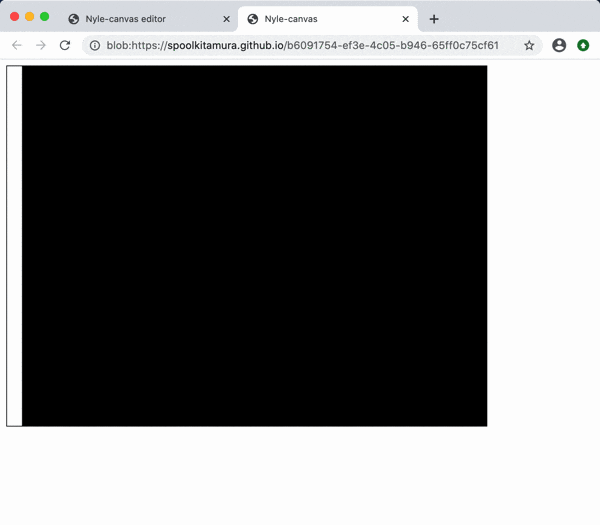### 2-2. Put out the wall (also on the right side) (rb_block02.html)

Next, make a vertical wall on the right side.

The `x` in the upper left corner is` 640 --20 = 620`, which is the window width 640 minus the wall thickness 20, and the `y` is` 0`.

The lower right corner is the same `(640, 480)` as the lower right corner of the window.

#### `rb_block02.rb`

``````
include DX
#Code for initial setting(your setup code here)
Window.width   = 640
Window.height  = 480
Window.bgcolor = C_BLACK

Window.loop do
Window.draw_box_fill(  0,   0,  20, 480, C_WHITE)   #◇ Change (character alignment)
Window.draw_box_fill(620,   0, 640, 480, C_WHITE)   #◆ Addition
end
``````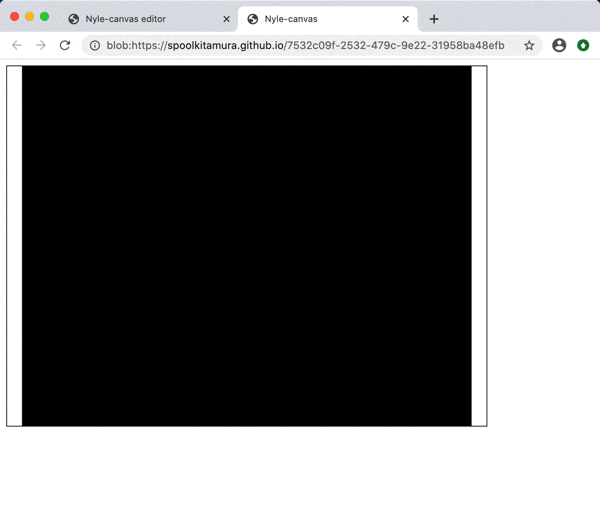### 2-3. Put out the wall (also on the upper side) (rb_block03.html)

Make the upper side wall (thickness 20).

The upper left corner is `(0, 0)`, the lower right corner `x` is the same as the window width` 640`, and `y` is the same as the wall thickness` 20`.

#### `rb_block03.rb`

``````
include DX
#Code for initial setting(your setup code here)
Window.width   = 640
Window.height  = 480
Window.bgcolor = C_BLACK

Window.loop do
Window.draw_box_fill(  0,   0,  20, 480, C_WHITE)
Window.draw_box_fill(620,   0, 640, 480, C_WHITE)
Window.draw_box_fill(  0,   0, 640,  20, C_WHITE)   #◆ Addition
end
``````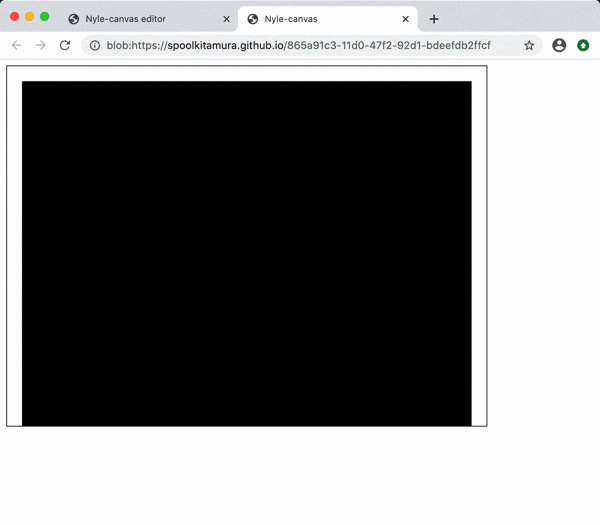### 2-4. Bring up the bar (rb_block04.html)

Prepare a light blue rectangular image (`src / image / bar.png`) with a width of 100 and a height of 20 as a bar to hit the ball back.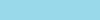First, drag and drop the `bar.png` file directly onto the editor screen. This will copy it into Nyle-canvas and display it in the image file list at the bottom of the editor screen.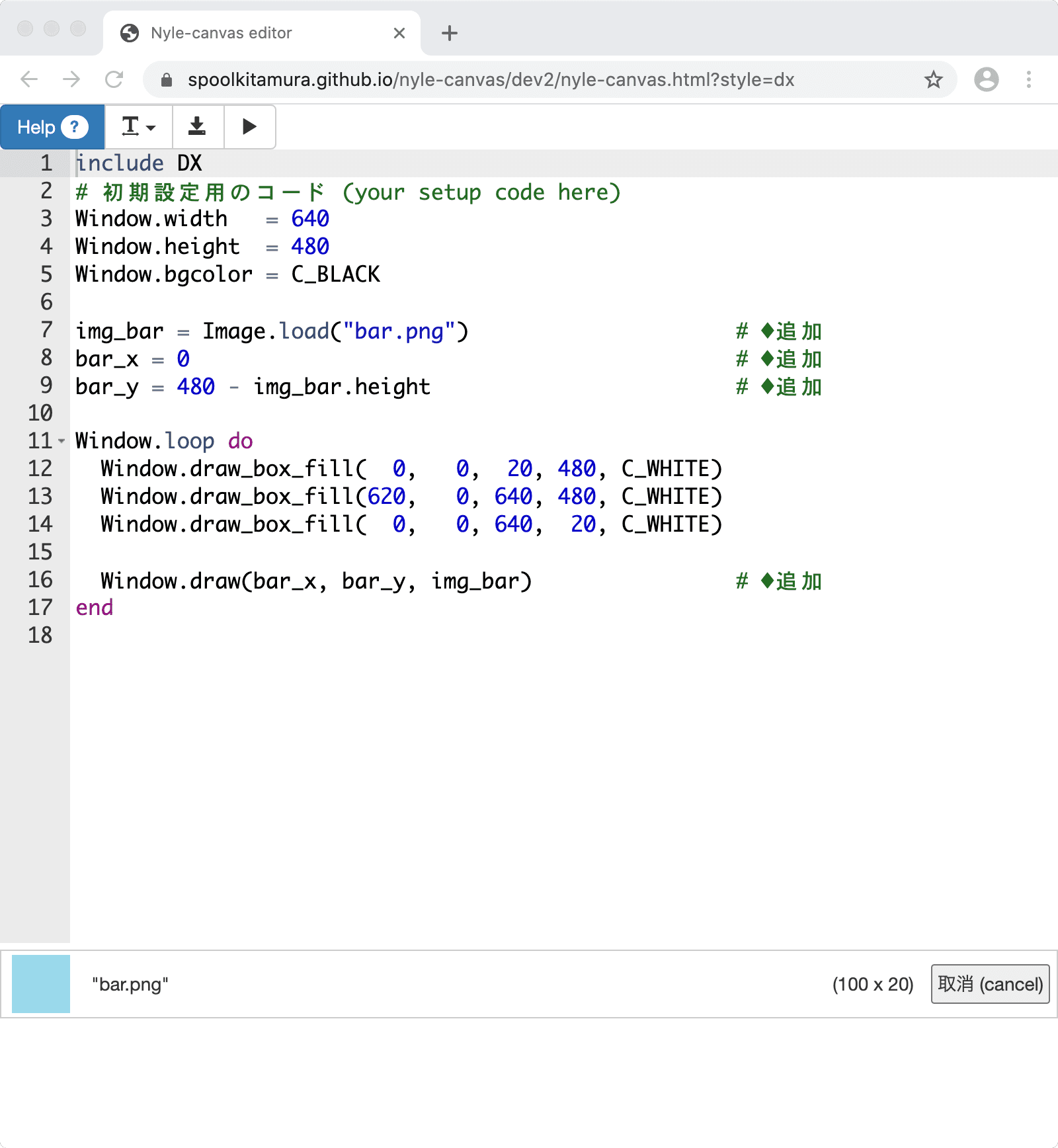Then load the image file with ʻImage.load ("bar.png ")`. (No path name is required.)

The vertical position of the bar, `y`, should be` 480 --the vertical width of the bar` to match the bottom of the window, `480`. The horizontal position `x` of the bar should be 0.

To display the bar, use `Window.draw (x position, y position, image)` in `Window.loop do ~ end`.

#### `rb_block04.rb`

``````
include DX
#Code for initial setting(your setup code here)
Window.width   = 640
Window.height  = 480
Window.bgcolor = C_BLACK

bar_y = 480 - img_bar.height                        #◆ Addition

Window.loop do
Window.draw_box_fill(  0,   0,  20, 480, C_WHITE)
Window.draw_box_fill(620,   0, 640, 480, C_WHITE)
Window.draw_box_fill(  0,   0, 640,  20, C_WHITE)

end
``````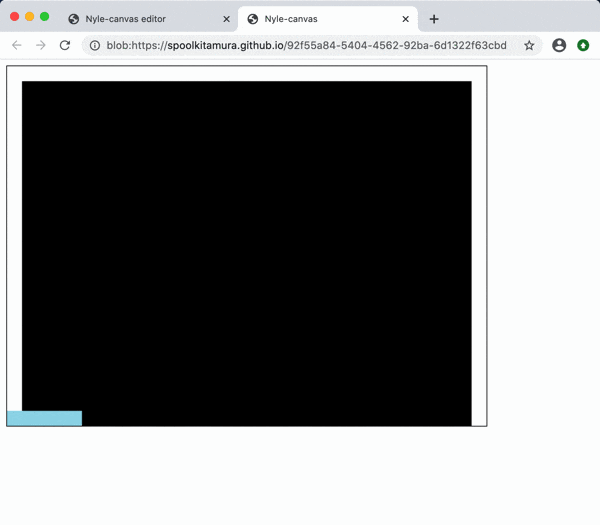### 2-5. Move the bar with the arrow keys (rb_block05.html)

Use the left and right arrow keys (`←` `→`) on the keyboard to move the bar left and right (`x direction`).

Move the bar by getting the pressed state of the left and right arrow keys with ʻInput.x` and adding the values (`-1`, `0`,` 1`) to the `x position` of the bar. I will.

#### `rb_block05.rb`

``````
include DX
#Code for initial setting(your setup code here)
Window.width   = 640
Window.height  = 480
Window.bgcolor = C_BLACK

bar_x = 0
bar_y = 480 - img_bar.height

Window.loop do
bar_x = bar_x + Input.x             #◆ Addition

Window.draw_box_fill(  0,   0,  20, 480, C_WHITE)
Window.draw_box_fill(620,   0, 640, 480, C_WHITE)
Window.draw_box_fill(  0,   0, 640,  20, C_WHITE)

Window.draw(bar_x, bar_y, img_bar)
end
``````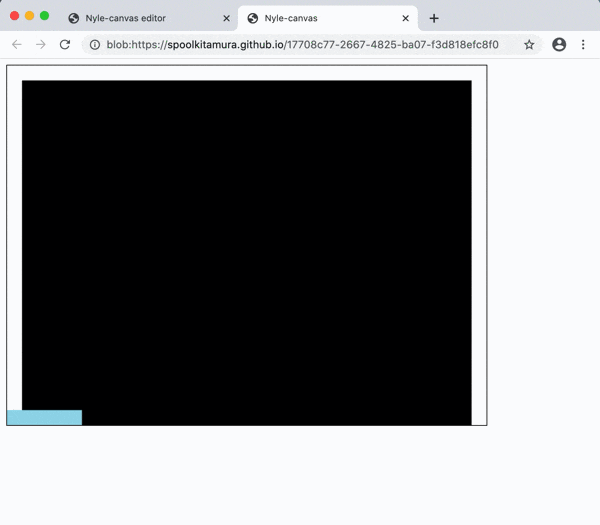### 2-6. Move the bar with the arrow keys: Another way to write (rb_block06.html)

Another way to write `bar_x = bar_x + Input.x`,

Let's rewrite it as `bar_x + = Input.x`. It's the same, but if you get used to it, it might be easier to see.

#### `rb_block06.rb`

``````
include DX
#Code for initial setting(your setup code here)
Window.width   = 640
Window.height  = 480
Window.bgcolor = C_BLACK

bar_x = 0
bar_y = 480 - img_bar.height

Window.loop do
bar_x += Input.x            #◇ Change (change the writing style)

Window.draw_box_fill(  0,   0,  20, 480, C_WHITE)
Window.draw_box_fill(620,   0, 640, 480, C_WHITE)
Window.draw_box_fill(  0,   0, 640,  20, C_WHITE)

Window.draw(bar_x, bar_y, img_bar)
end
``````

### 2-7. Quickly move the bar (rb_block07.html)

Let's move the bar quickly.

ʻInput.x` returns only the values` -1`, `0`,` 1`, so I'll multiply it by 4 to increase it and add it to the `x position` of the bar.

#### `rb_block07.rb`

``````
include DX
#Code for initial setting(your setup code here)
Window.width   = 640
Window.height  = 480
Window.bgcolor = C_BLACK

bar_x = 0
bar_y = 480 - img_bar.height

Window.loop do
bar_x += Input.x * 4        #◇ Change (faster reaction)

Window.draw_box_fill(  0,   0,  20, 480, C_WHITE)
Window.draw_box_fill(620,   0, 640, 480, C_WHITE)
Window.draw_box_fill(  0,   0, 640,  20, C_WHITE)

Window.draw(bar_x, bar_y, img_bar)
end
``````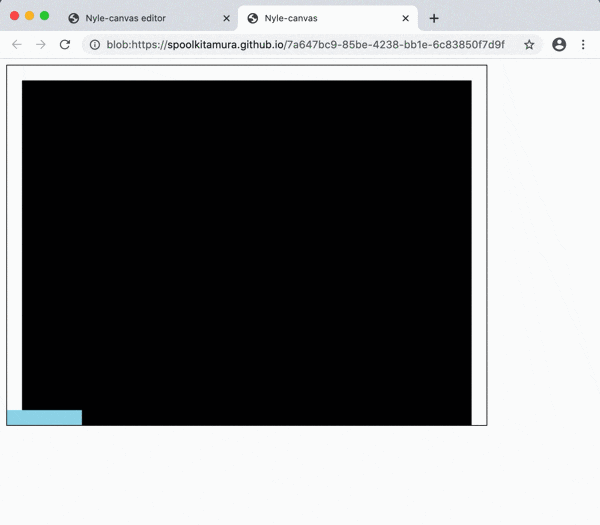### 2-8. Move the bar with the mouse (rb_block08.html)

Makes the bar move left and right (`x direction`) following the movement of the mouse.

Move the bar by getting the `x position` of the mouse with ʻInput.mouse_x` and assigning that value to the` x position` of the bar.

• The `bar` can be moved with the` left / right key` or with the `mouse`, but from this point onward, it will be shown using the` mouse`.

#### `rb_block08.rb`

``````
include DX
#Code for initial setting(your setup code here)
Window.width   = 640
Window.height  = 480
Window.bgcolor = C_BLACK

bar_x = 0
bar_y = 480 - img_bar.height

Window.loop do
#bar_x += Input.x * 4       #For left and right keys#◇ Change (comment out)
bar_x = Input.mouse_x       #For mouse#◆ Addition

Window.draw_box_fill(  0,   0,  20, 480, C_WHITE)
Window.draw_box_fill(620,   0, 640, 480, C_WHITE)
Window.draw_box_fill(  0,   0, 640,  20, C_WHITE)

Window.draw(bar_x, bar_y, img_bar)
end
``````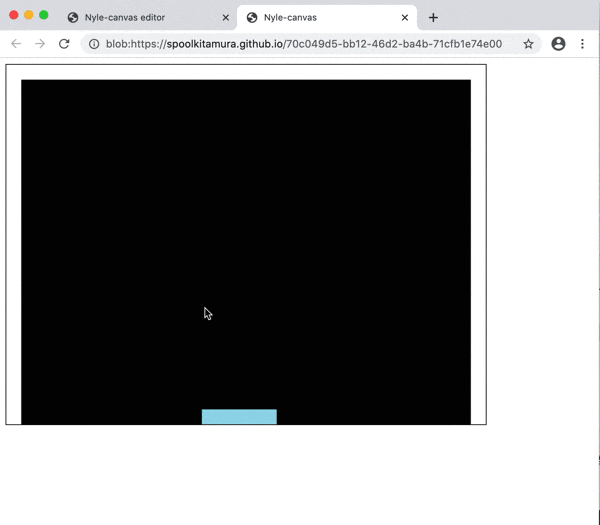### 2-9. Do not let the bar stick out (rb_block09.html)

Make sure the bar does not stick out of the left and right walls.

The `x position` of the bar is on the far left, so the minimum value is the wall thickness` 20`.

On the other hand, the right edge is `x position + bar width img_bar.width`, so the maximum value is` window width 640 --wall thickness 20 --bar width img_bar.width`.

Write the above in ʻif ~ elsif ~ end`.

#### `rb_block09.rb`

``````
include DX
#Code for initial setting(your setup code here)
Window.width   = 640
Window.height  = 480
Window.bgcolor = C_BLACK

bar_x = 0
bar_y = 480 - img_bar.height

Window.loop do
#bar_x += Input.x * 4       #For left and right keys
bar_x = Input.mouse_x       #For mouse
if bar_x < 20                              #◆ Addition
elsif bar_x > 640 - 20 - img_bar.width     #◆ Addition
bar_x = 640 - 20 - img_bar.width         #◆ Addition

Window.draw_box_fill(  0,   0,  20, 480, C_WHITE)
Window.draw_box_fill(620,   0, 640, 480, C_WHITE)
Window.draw_box_fill(  0,   0, 640,  20, C_WHITE)

Window.draw(bar_x, bar_y, img_bar)
end
``````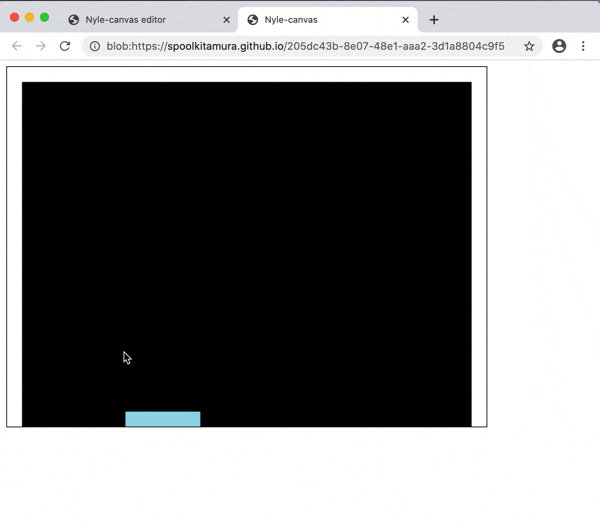### 2-10. Put out the ball (rb_block10.html)

Prepare a red circle image (`src / image / ball.png`) with 20 horizontal and 20 vertical as a ball.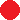Drag and drop the `ball.png` file onto the editor screen, register it in Nyle-canvas, and use ʻImage.load (" ball.png ")` in the same way as in "2-4. Bring out the bar". Read.

#### Note) About transparency of images

In `dev2` released on May 30, 2020, the white color of the image is automatically made transparent at the time of ʻImage.load`. Therefore, the background of `ball.png`, which has a red ball drawn on a white background, is automatically transparent.

For the time being, let the x position be `ball_x = 300` and the y position be` ball_y = 400`.

After that, draw with `Window.draw`.

#### `rb_block10.rb`

``````
include DX
#Code for initial setting(your setup code here)
Window.width   = 640
Window.height  = 480
Window.bgcolor = C_BLACK

bar_x = 0
bar_y = 480 - img_bar.height

Window.loop do
#bar_x += Input.x * 4       #For left and right keys
bar_x = Input.mouse_x       #For mouse
if bar_x < 20
bar_x = 20
elsif bar_x > 640 - 20 - img_bar.width
bar_x = 640 - 20 - img_bar.width
end

Window.draw_box_fill(  0,   0,  20, 480, C_WHITE)
Window.draw_box_fill(620,   0, 640, 480, C_WHITE)
Window.draw_box_fill(  0,   0, 640,  20, C_WHITE)

Window.draw(bar_x, bar_y, img_bar)
end
``````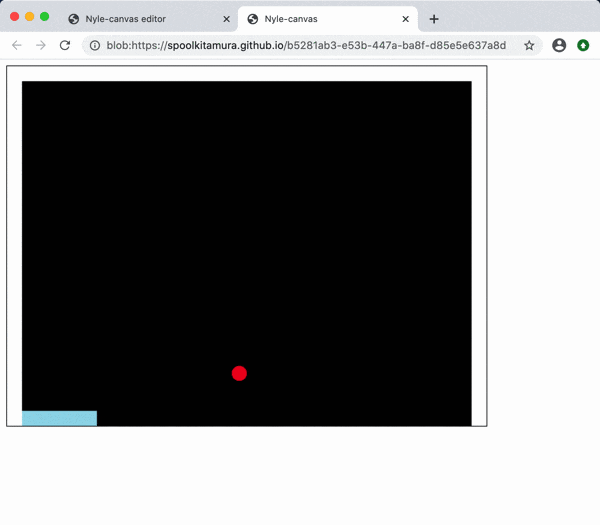### 2-11. Move the ball (horizontal direction) (rb_block11.html)

Move the ball laterally (`x direction`). (`X` is in the plus direction on the right)

With the speed in the x direction as `dx`, add` dx` to the x position `ball_x` of the ball every time it turns in a loop (1/60 seconds) (` ball_x + = dx`).

#### `rb_block11.rb`

``````
include DX
#Code for initial setting(your setup code here)
Window.width   = 640
Window.height  = 480
Window.bgcolor = C_BLACK

bar_x = 0
bar_y = 480 - img_bar.height

ball_x = 300
ball_y = 400

Window.loop do
#bar_x += Input.x * 4       #For left and right keys
bar_x = Input.mouse_x       #For mouse
if bar_x < 20
bar_x = 20
elsif bar_x > 640 - 20 - img_bar.width
bar_x = 640 - 20 - img_bar.width
end

Window.draw_box_fill(  0,   0,  20, 480, C_WHITE)
Window.draw_box_fill(620,   0, 640, 480, C_WHITE)
Window.draw_box_fill(  0,   0, 640,  20, C_WHITE)

Window.draw(bar_x, bar_y, img_bar)
Window.draw(ball_x, ball_y, img_ball)
end
``````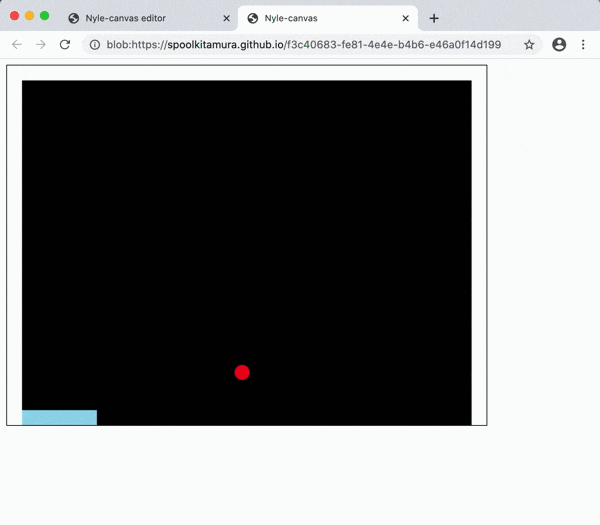### 2-12. Move the ball (vertical) (rb_block12.html)

Now move it vertically (`y direction`). (The bottom of `y` is in the plus direction)

Stop moving it horizontally (comment out with `# ball_x + = dx`), set the speed in the y direction to` dy`, and add `dy` every time it turns in a loop (` ball_y + = dy`).

#### `rb_block12.rb`

``````
include DX
#Code for initial setting(your setup code here)
Window.width   = 640
Window.height  = 480
Window.bgcolor = C_BLACK

bar_x = 0
bar_y = 480 - img_bar.height

ball_x = 300
ball_y = 400
dx     =   2       #◇ Change (character alignment)

Window.loop do
#bar_x += Input.x * 4       #For left and right keys
bar_x = Input.mouse_x       #For mouse
if bar_x < 20
bar_x = 20
elsif bar_x > 640 - 20 - img_bar.width
bar_x = 640 - 20 - img_bar.width
end

#ball_x += dx                 #◇ Change (comment out)

Window.draw_box_fill(  0,   0,  20, 480, C_WHITE)
Window.draw_box_fill(620,   0, 640, 480, C_WHITE)
Window.draw_box_fill(  0,   0, 640,  20, C_WHITE)

Window.draw(bar_x, bar_y, img_bar)
Window.draw(ball_x, ball_y, img_ball)
end
``````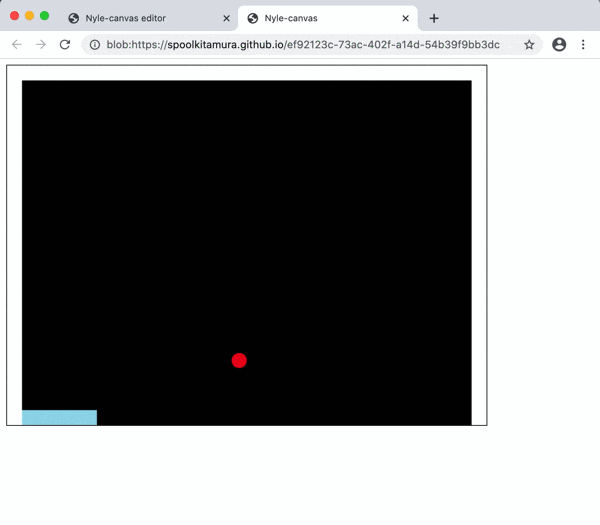### 2-13. Move the ball (vertical and horizontal) (rb_block13.html)

If you resume the movement in the `x direction` (uncomment out` ball_x + = dx`), the ball will move diagonally.

#### `rb_block13.rb`

``````
include DX
#Code for initial setting(your setup code here)
Window.width   = 640
Window.height  = 480
Window.bgcolor = C_BLACK

bar_x = 0
bar_y = 480 - img_bar.height

ball_x = 300
ball_y = 400
dx     =   2
dy     =  -2

Window.loop do
#bar_x += Input.x * 4       #For left and right keys
bar_x = Input.mouse_x       #For mouse
if bar_x < 20
bar_x = 20
elsif bar_x > 640 - 20 - img_bar.width
bar_x = 640 - 20 - img_bar.width
end

ball_x += dx                 #◇ Change (uncomment)
ball_y += dy

Window.draw_box_fill(  0,   0,  20, 480, C_WHITE)
Window.draw_box_fill(620,   0, 640, 480, C_WHITE)
Window.draw_box_fill(  0,   0, 640,  20, C_WHITE)

Window.draw(bar_x, bar_y, img_bar)
Window.draw(ball_x, ball_y, img_ball)
end
``````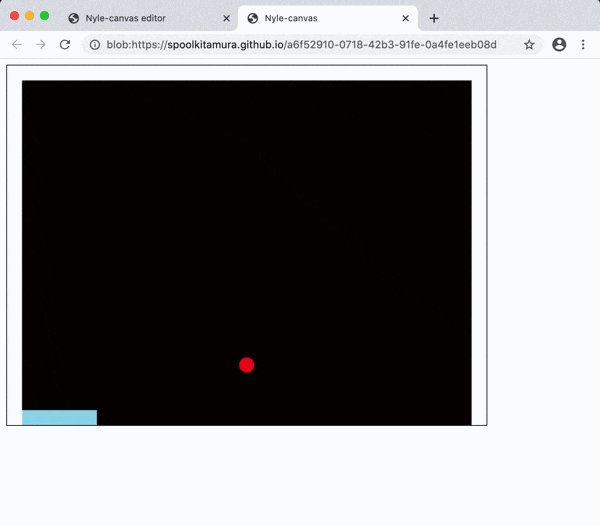### 2-14. The ball bounces (horizontally) (rb_block14.html)

Make the ball bounce. First, move it only in the horizontal direction (`x direction`) (comment out with` # ball_y + = dy`).

The hit on the left wall is when the `ball x position` (` ball_x`) is smaller than the left wall thickness of `20`.

On the other hand, did you hit the right wall when the width of the window was 640-the thickness of the right wall was 20 = 620`?

Bounce means that the speed `dx` in the x direction is in the opposite direction, so you can write` dx = -dx`.

I will write the above in ʻif ~ end`.

#### `rb_block14.rb`

``````
include DX
#Code for initial setting(your setup code here)
Window.width   = 640
Window.height  = 480
Window.bgcolor = C_BLACK

bar_x = 0
bar_y = 480 - img_bar.height

img_ball.set_color_key(C_WHITE)
ball_x = 300
ball_y = 400
dx     =   2
dy     =  -2

Window.loop do
#bar_x += Input.x * 4       #For left and right keys
bar_x = Input.mouse_x       #For mouse
if bar_x < 20
bar_x = 20
elsif bar_x > 640 - 20 - img_bar.width
bar_x = 640 - 20 - img_bar.width
end

ball_x += dx
#ball_y += dy                     #◇ Change (comment out)

if ball_x < 20 || ball_x > 620    #◆ Addition
include DX
#Code for initial setting(your setup code here)
Window.width   = 640
Window.height  = 480
Window.bgcolor = C_BLACK

bar_x = 0
bar_y = 480 - img_bar.height

ball_x = 300
ball_y = 400
dx     =   2
dy     =  -2

Window.loop do
#bar_x += Input.x * 4       #For left and right keys
bar_x = Input.mouse_x       #For mouse
if bar_x < 20
bar_x = 20
elsif bar_x > 640 - 20 - img_bar.width
bar_x = 640 - 20 - img_bar.width
end

ball_x += dx
#ball_y += dy                     #◇ Change (comment out)

if ball_x < 20 || ball_x > 620    #◆ Addition

Window.draw_box_fill(  0,   0,  20, 480, C_WHITE)
Window.draw_box_fill(620,   0, 640, 480, C_WHITE)
Window.draw_box_fill(  0,   0, 640,  20, C_WHITE)

Window.draw(bar_x, bar_y, img_bar)
Window.draw(ball_x, ball_y, img_ball)
end
``````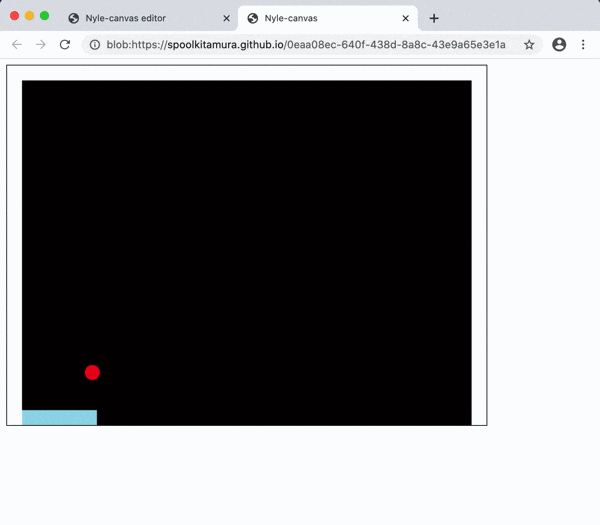The right side has gone into the wall.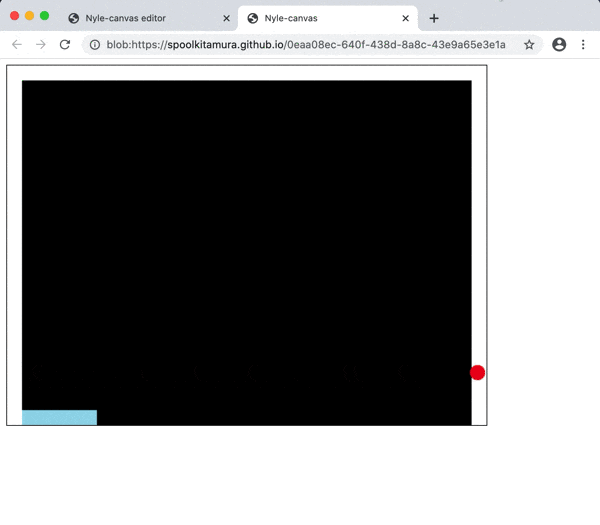### 2-15. Ball bounces (horizontal): modified (rb_block15.html)

The `ball x position` (` ball_x`) is the left edge of the ball, so the right edge is `ball_x + ball_width`, which is the sum of the` ball width` (` ball_width`).

"Hit the right wall" must be when this value is greater than `window width 640 --right wall thickness 20 = 620`.

`(ball_x + ball_width) > 620`

In addition, in order to slowly observe the state of the collision, the setting of the number of drawings per second (initial value: 60) is halved to 30.

`Window.fps = 30`

#### `rb_block15.rb`

``````
include DX
#Code for initial setting(your setup code here)
Window.width   = 640
Window.height  = 480
Window.bgcolor = C_BLACK

bar_x = 0
bar_y = 480 - img_bar.height

ball_x = 300
ball_y = 400
dx     =   2
dy     =  -2

#Setting the number of drawings per second (initial value: 60)#◆ Addition

Window.loop do
#bar_x += Input.x * 4       #For left and right keys
bar_x = Input.mouse_x       #For mouse
if bar_x < 20
bar_x = 20
elsif bar_x > 640 - 20 - img_bar.width
bar_x = 640 - 20 - img_bar.width
end

ball_x += dx
#ball_y += dy

if ball_x < 20 || (ball_x + ball_width) > 620   #◇ Change
dx = -dx
end

Window.draw_box_fill(  0,   0,  20, 480, C_WHITE)
Window.draw_box_fill(620,   0, 640, 480, C_WHITE)
Window.draw_box_fill(  0,   0, 640,  20, C_WHITE)

Window.draw(bar_x, bar_y, img_bar)
Window.draw(ball_x, ball_y, img_ball)
end
``````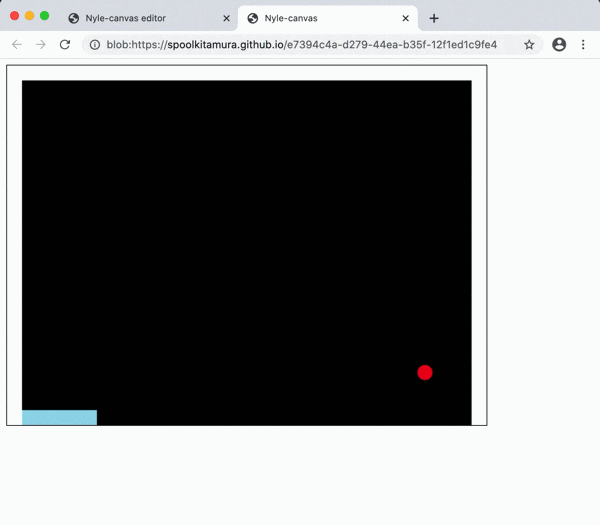This looks good, but when you look closely, the ball is sunk into the wall for a moment (both the left and right walls).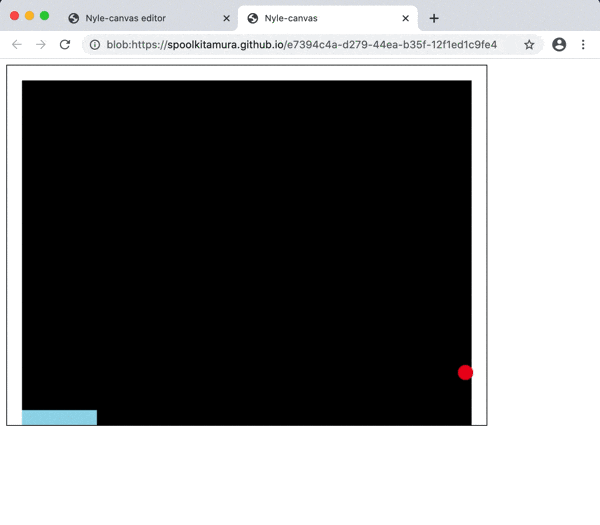### 2-16. Ball bounces (horizontal): Fix 2 (rb_block16.html)

Previously, if you hit a wall, you would just reverse the velocity of the ball in the `x direction` (` dx = -dx`).

This will bounce, but the position of the ball (`ball_x`) hasn't changed, so the ball remains stuck in the wall.

Therefore, the position of the ball (`ball_x`) will also be reversed by the amount of movement (`+ dx`) (`ball_x-= dx`).

#### `rb_block16.rb`

``````
include DX
#Code for initial setting(your setup code here)
Window.width   = 640
Window.height  = 480
Window.bgcolor = C_BLACK

bar_x = 0
bar_y = 480 - img_bar.height

ball_x = 300
ball_y = 400
dx     =   2
dy     =  -2
ball_width  = img_ball.width

#Setting the number of drawings per second (initial value: 60)
Window.fps = 30

Window.loop do
#bar_x += Input.x * 4       #For left and right keys
bar_x = Input.mouse_x       #For mouse
if bar_x < 20
bar_x = 20
elsif bar_x > 640 - 20 - img_bar.width
bar_x = 640 - 20 - img_bar.width
end

ball_x += dx
#ball_y += dy

if ball_x < 20 || (ball_x + ball_width) > 620
dx = -dx
end

Window.draw_box_fill(  0,   0,  20, 480, C_WHITE)
Window.draw_box_fill(620,   0, 640, 480, C_WHITE)
Window.draw_box_fill(  0,   0, 640,  20, C_WHITE)

Window.draw(bar_x, bar_y, img_bar)
Window.draw(ball_x, ball_y, img_ball)
end
``````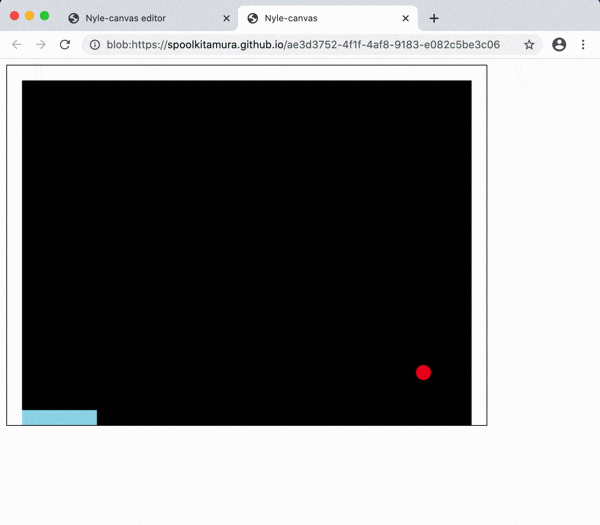It looks good here.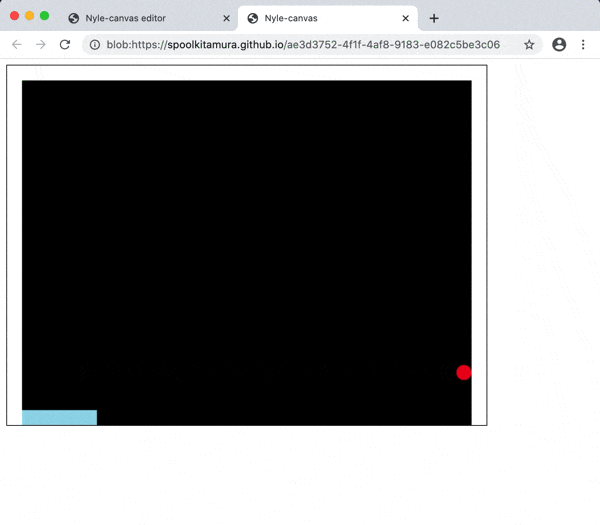### 2-17. The ball bounces (vertical) (rb_block17.html)

Now move the ball only vertically (`y direction`) so that it bounces off the top wall.

The hit on the upper wall is when the `y position` (` ball_y`) of the ball becomes smaller than the thickness `20` of the upper wall.

In addition, the number of drawing times per second is restored (commented out), and the initial value of the `x position` of the bar is changed toward the center.

#### `rb_block17.rb`

``````
include DX
#Code for initial setting(your setup code here)
Window.width   = 640
Window.height  = 480
Window.bgcolor = C_BLACK

bar_x = 250                        #◇ Change (numerical change)
bar_y = 480 - img_bar.height

ball_x = 300
ball_y = 400
dx     =   2
dy     =  -2
ball_width  = img_ball.width

#Setting the number of drawings per second (initial value: 60)
#Window.fps = 30                    #◇ Change (comment out)

Window.loop do
#bar_x += Input.x * 4       #For left and right keys
bar_x = Input.mouse_x       #For mouse
if bar_x < 20
bar_x = 20
elsif bar_x > 640 - 20 - img_bar.width
bar_x = 640 - 20 - img_bar.width
end

#ball_x += dx                     #◇ Change (comment out)
ball_y += dy                      #◇ Change (uncomment)

if ball_x < 20 || (ball_x + ball_width) > 620
ball_x -= dx
dx = -dx
end

if ball_y < 20                   #◆ Addition

Window.draw_box_fill(  0,   0,  20, 480, C_WHITE)
Window.draw_box_fill(620,   0, 640, 480, C_WHITE)
Window.draw_box_fill(  0,   0, 640,  20, C_WHITE)

Window.draw(bar_x, bar_y, img_bar)
Window.draw(ball_x, ball_y, img_ball)
end
``````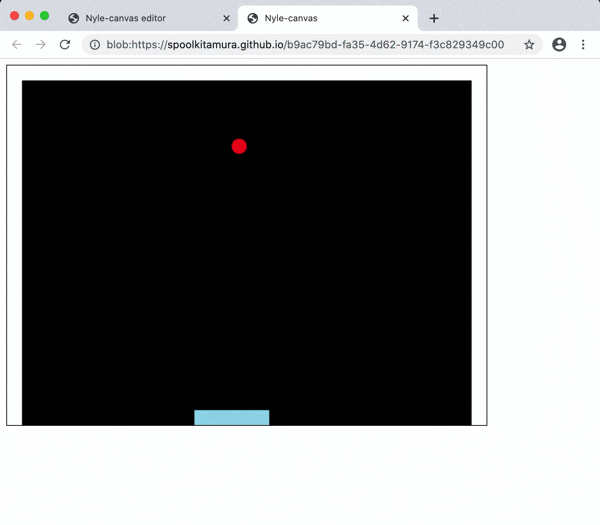It still doesn't bounce off the bar.

### 2-18. The ball bounces (vertical and horizontal) (rb_block18.html)

Move it vertically and horizontally to make it bounce. The atmosphere of "breakout" has come out a little.

#### `rb_block18.rb`

``````
include DX
#Code for initial setting(your setup code here)
Window.width   = 640
Window.height  = 480
Window.bgcolor = C_BLACK

bar_x = 250
bar_y = 480 - img_bar.height

ball_x = 300
ball_y = 400
dx     =   2
dy     =  -2
ball_width  = img_ball.width
ball_height = img_ball.height

#Setting the number of drawings per second (initial value: 60)
#Window.fps = 30

Window.loop do
#bar_x += Input.x * 4       #For left and right keys
bar_x = Input.mouse_x       #For mouse
if bar_x < 20
bar_x = 20
elsif bar_x > 640 - 20 - img_bar.width
bar_x = 640 - 20 - img_bar.width
end

ball_x += dx                   #◇ Change (uncomment)
ball_y += dy

if ball_x < 20 || (ball_x + ball_width) > 620
ball_x -= dx
dx = -dx
end

if ball_y < 20
ball_y -= dy
dy = -dy
end

Window.draw_box_fill(  0,   0,  20, 480, C_WHITE)
Window.draw_box_fill(620,   0, 640, 480, C_WHITE)
Window.draw_box_fill(  0,   0, 640,  20, C_WHITE)

Window.draw(bar_x, bar_y, img_bar)
Window.draw(ball_x, ball_y, img_ball)
end
``````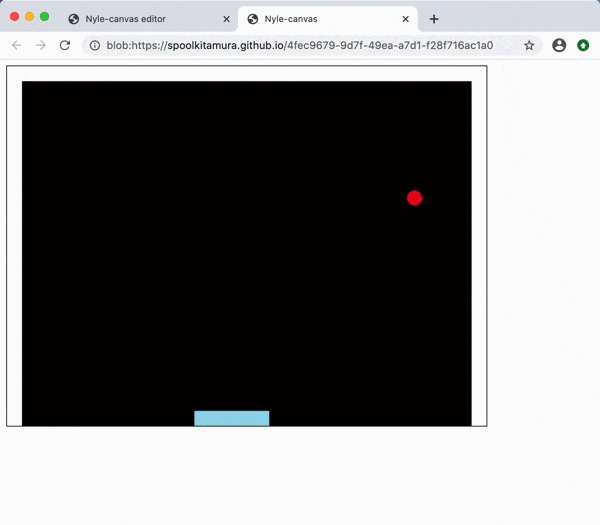### 2-19. The ball bounces (with the bar) (rb_block19.html)

Try to bounce at the bar as well.

The conditions when the ball hits the bar are;

#### ○ `y coordinate`

--` Below the ball`>` Above the bar`

`（ball_y + ball_height） > (480 - bar_height)`

#### ○ `x coordinates`

--`Right of the ball`> `Left of the bar`

`(ball_x + ball_width) > bar_x`

--`Left of the ball` <`Right of the bar`

`ball_x < (bar_x + bar_width)`

It is time to meet the above conditions at the same time (`&&`).

#### `rb_block19.rb`

``````
include DX
#Code for initial setting(your setup code here)
Window.width   = 640
Window.height  = 480
Window.bgcolor = C_BLACK

bar_x = 250
bar_y = 480 - img_bar.height

ball_x = 300
ball_y = 400
dx     =   2
dy     =  -2
ball_width  = img_ball.width
ball_height = img_ball.height

#Setting the number of drawings per second (initial value: 60)
#Window.fps = 30

Window.loop do
#bar_x += Input.x * 4       #For left and right keys
bar_x = Input.mouse_x       #For mouse
if bar_x < 20
bar_x = 20
elsif bar_x > 640 - 20 - img_bar.width
bar_x = 640 - 20 - img_bar.width
end

ball_x += dx
ball_y += dy

if ball_x < 20 || (ball_x + ball_width) > 620
ball_x -= dx
dx = -dx
end

if ball_y < 20
ball_y -= dy
dy = -dy
end

if (ball_y + ball_height) > (480 - bar_height)  &&  #◆ Addition
(ball_x + ball_width)  > bar_x               &&  #◆ Addition
ball_x                 < (bar_x + bar_width)     #◆ Addition

Window.draw_box_fill(  0,   0,  20, 480, C_WHITE)
Window.draw_box_fill(620,   0, 640, 480, C_WHITE)
Window.draw_box_fill(  0,   0, 640,  20, C_WHITE)

Window.draw(bar_x, bar_y, img_bar)
Window.draw(ball_x, ball_y, img_ball)
end
``````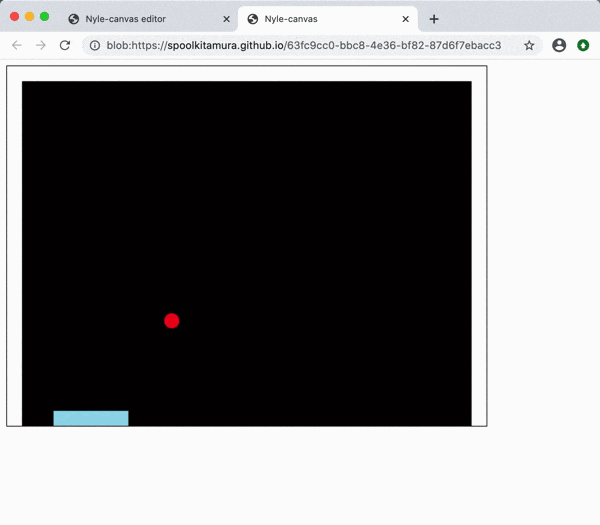This looks good, but the bounce when hit from the side while moving the bar is strange.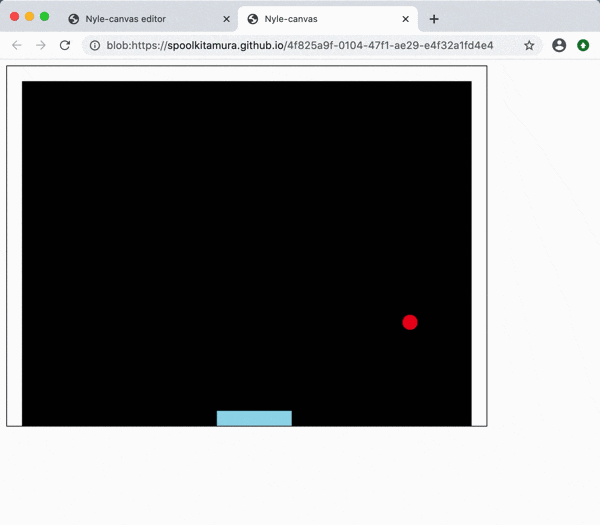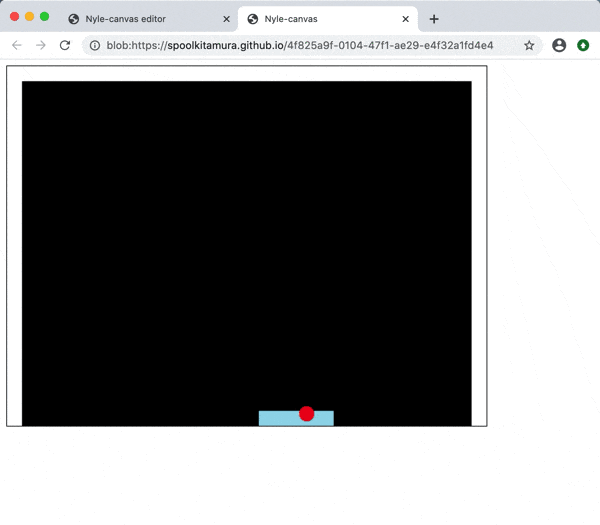### 2-20. Ball bounces (with bar): fix (rb_block20.html)

Once the ball gets too far into the bar, it's still inside the bar even after the bounce process, so the `dy` just repeats plus and minus and you can't get out of the bar.

So, add a condition that `bounces only if you can get out of the bar when you're bounced'.

In the `y direction`

`Under the ball` <=` Below the bar by the absolute value of dy`

`(ball_y + ball_height) <= (480 - bar_height + dy.abs)`

#### `rb_block20.rb`

``````
include DX
#Code for initial setting(your setup code here)
Window.width   = 640
Window.height  = 480
Window.bgcolor = C_BLACK

bar_x = 250
bar_y = 480 - img_bar.height
bar_width  = img_bar.width
bar_height = img_bar.height

ball_x = 300
ball_y = 400
dx     =   2
dy     =  -2
ball_width  = img_ball.width
ball_height = img_ball.height

#Setting the number of drawings per second (initial value: 60)
#Window.fps = 30

Window.loop do
#bar_x += Input.x * 4       #For left and right keys
bar_x = Input.mouse_x       #For mouse
if bar_x < 20
bar_x = 20
elsif bar_x > 640 - 20 - img_bar.width
bar_x = 640 - 20 - img_bar.width
end

ball_x += dx
ball_y += dy

if ball_x < 20 || (ball_x + ball_width) > 620
ball_x -= dx
dx = -dx
end

if ball_y < 20
ball_y -= dy
dy = -dy
end

if (ball_y + ball_height) >  (480 - bar_height)  &&           #◇ Change (character alignment)
(ball_y + ball_height) <= (480 - bar_height + dy.abs)  &&  #◆ Addition
(ball_x + ball_width)  >  bar_x               &&           #◇ Change (character alignment)
ball_x                 <  (bar_x + bar_width)              #◇ Change (character alignment)

ball_y -= dy
dy = -dy
end

Window.draw_box_fill(  0,   0,  20, 480, C_WHITE)
Window.draw_box_fill(620,   0, 640, 480, C_WHITE)
Window.draw_box_fill(  0,   0, 640,  20, C_WHITE)

Window.draw(bar_x, bar_y, img_bar)
Window.draw(ball_x, ball_y, img_ball)
end
``````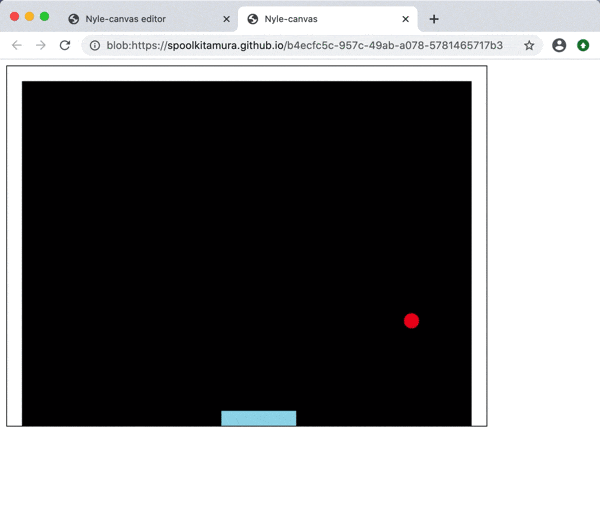This time it looks good.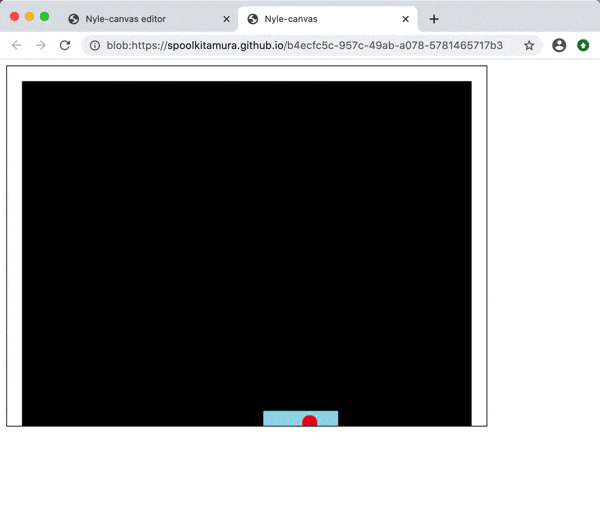### 2-21. Put out a block (1) (rb_block21.html)

From here, we will make blocks. First one.

Prepare a green rectangular image (`src / image / block.png`) with a size of 58 horizontal and 18 vertical as a block.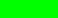The width is set to `(window width 640 --thickness of left and right walls 20 * 2) / 10 = 60`, and the gap between the neighbors is set to` 58` by taking 1 each on the left and right. ..

Drag and drop this `block.png` file onto the editor screen and register it in Nyle-canvas.

Then load the image file with ʻImage.load ("block.png ")`. (No path name is required.)

With the first block as `block00`, the position is from the inside of the upper left wall (`x = 20`, `y = 20`) with a gap of` 1` in both horizontal and vertical directions, `block00_x = 21`,` Place it at block00_y = 21`.

Then draw with `Window.draw (block00_x, block00_y, img_block)`.

#### `rb_block21.rb`

``````
include DX
#Code for initial setting(your setup code here)
Window.width   = 640
Window.height  = 480
Window.bgcolor = C_BLACK

bar_x = 250
bar_y = 480 - img_bar.height
bar_width  = img_bar.width
bar_height = img_bar.height

ball_x = 300
ball_y = 400
dx     =   2
dy     =  -2
ball_width  = img_ball.width
ball_height = img_ball.height

#Setting the number of drawings per second (initial value: 60)
#Window.fps = 30

Window.loop do
#bar_x += Input.x * 4       #For left and right keys
bar_x = Input.mouse_x       #For mouse
if bar_x < 20
bar_x = 20
elsif bar_x > 640 - 20 - img_bar.width
bar_x = 640 - 20 - img_bar.width
end

ball_x += dx
ball_y += dy

if ball_x < 20 || (ball_x + ball_width) > 620
ball_x -= dx
dx = -dx
end

if ball_y < 20
ball_y -= dy
dy = -dy
end

if (ball_y + ball_height) >  (480 - bar_height)  &&
(ball_y + ball_height) <= (480 - bar_height + dy.abs)  &&
(ball_x + ball_width)  >  bar_x               &&
ball_x                 <  (bar_x + bar_width)

ball_y -= dy
dy = -dy
end

Window.draw_box_fill(  0,   0,  20, 480, C_WHITE)
Window.draw_box_fill(620,   0, 640, 480, C_WHITE)
Window.draw_box_fill(  0,   0, 640,  20, C_WHITE)

Window.draw(bar_x, bar_y, img_bar)
Window.draw(ball_x, ball_y, img_ball)

end
``````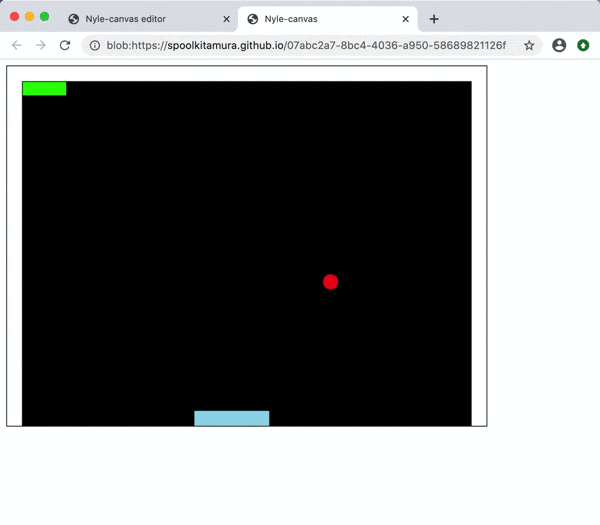### 2-22. Put out blocks (2) (rb_block22.html)

The second block (`block01`) uses the same image and is shifted to the right by`block width (block_widh) + gap (2)`.

#### `rb_block22.rb`

``````
include DX
#Code for initial setting(your setup code here)
Window.width   = 640
Window.height  = 480
Window.bgcolor = C_BLACK

bar_x = 250
bar_y = 480 - img_bar.height
bar_width  = img_bar.width
bar_height = img_bar.height

ball_x = 300
ball_y = 400
dx     =   2
dy     =  -2
ball_width  = img_ball.width
ball_height = img_ball.height

block_widh = img_block.width
block_height = img_block.height

block00_x = 21
block00_y = 21

block01_x = 21 + block_widh + 2                    #◆ Addition

#Setting the number of drawings per second (initial value: 60)
#Window.fps = 30

Window.loop do
#bar_x += Input.x * 4       #For left and right keys
bar_x = Input.mouse_x       #For mouse
if bar_x < 20
bar_x = 20
elsif bar_x > 640 - 20 - img_bar.width
bar_x = 640 - 20 - img_bar.width
end

ball_x += dx
ball_y += dy

if ball_x < 20 || (ball_x + ball_width) > 620
ball_x -= dx
dx = -dx
end

if ball_y < 20
ball_y -= dy
dy = -dy
end

if (ball_y + ball_height) >  (480 - bar_height)  &&
(ball_y + ball_height) <= (480 - bar_height + dy.abs)  &&
(ball_x + ball_width)  >  bar_x               &&
ball_x                 <  (bar_x + bar_width)

ball_y -= dy
dy = -dy
end

Window.draw_box_fill(  0,   0,  20, 480, C_WHITE)
Window.draw_box_fill(620,   0, 640, 480, C_WHITE)
Window.draw_box_fill(  0,   0, 640,  20, C_WHITE)

Window.draw(bar_x, bar_y, img_bar)
Window.draw(ball_x, ball_y, img_ball)

Window.draw(block00_x, block00_y, img_block)
end
``````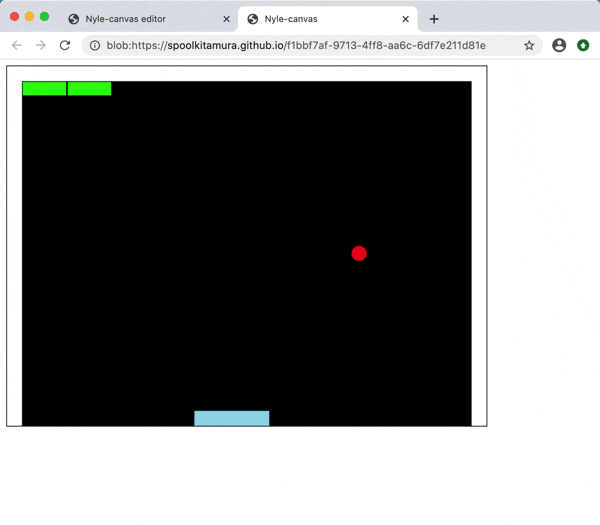### 2-23. Put out blocks (3) (rb_block23.html)

Also place the third block (block02). The x position is shifted by `block_widh + 2` in anticipation of the block and the gap.

#### `rb_block23.rb`

``````
include DX
#Code for initial setting(your setup code here)
Window.width   = 640
Window.height  = 480
Window.bgcolor = C_BLACK

bar_x = 250
bar_y = 480 - img_bar.height
bar_width  = img_bar.width
bar_height = img_bar.height

ball_x = 300
ball_y = 400
dx     =   2
dy     =  -2
ball_width  = img_ball.width
ball_height = img_ball.height

block_widh = img_block.width
block_height = img_block.height

block00_x = 21
block00_y = 21

block01_x = 21 + block_widh + 2
block01_y = 21

block02_x = 21 + (block_widh + 2) * 2              #◆ Addition

#Setting the number of drawings per second (initial value: 60)
#Window.fps = 30

Window.loop do
#bar_x += Input.x * 4       #For left and right keys
bar_x = Input.mouse_x       #For mouse
if bar_x < 20
bar_x = 20
elsif bar_x > 640 - 20 - img_bar.width
bar_x = 640 - 20 - img_bar.width
end

ball_x += dx
ball_y += dy

if ball_x < 20 || (ball_x + ball_width) > 620
ball_x -= dx
dx = -dx
end

if ball_y < 20
ball_y -= dy
dy = -dy
end

if (ball_y + ball_height) >  (480 - bar_height)  &&
(ball_y + ball_height) <= (480 - bar_height + dy.abs)  &&
(ball_x + ball_width)  >  bar_x               &&
ball_x                 <  (bar_x + bar_width)

ball_y -= dy
dy = -dy
end

Window.draw_box_fill(  0,   0,  20, 480, C_WHITE)
Window.draw_box_fill(620,   0, 640, 480, C_WHITE)
Window.draw_box_fill(  0,   0, 640,  20, C_WHITE)

Window.draw(bar_x, bar_y, img_bar)
Window.draw(ball_x, ball_y, img_ball)

Window.draw(block00_x, block00_y, img_block)
Window.draw(block01_x, block01_y, img_block)
end
``````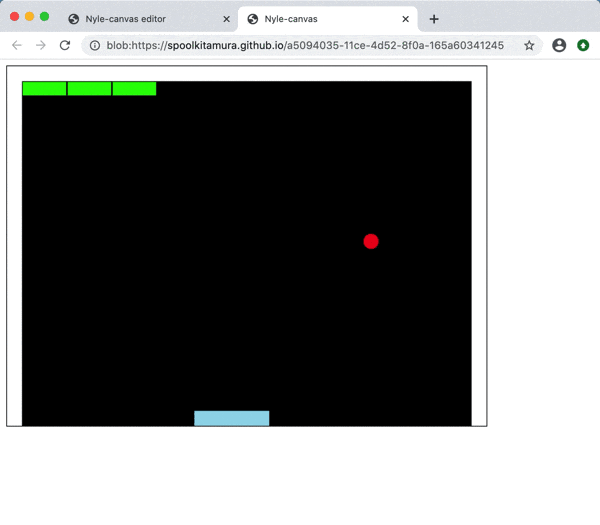### 2-24. Put out blocks (10) (rb_block24.html)

If you put 10 on the side, it's just the right width.

#### `rb_block24.rb`

``````
include DX
#Code for initial setting(your setup code here)
Window.width   = 640
Window.height  = 480
Window.bgcolor = C_BLACK

bar_x = 250
bar_y = 480 - img_bar.height
bar_width  = img_bar.width
bar_height = img_bar.height

ball_x = 300
ball_y = 400
dx     =   2
dy     =  -2
ball_width  = img_ball.width
ball_height = img_ball.height

block_widh = img_block.width
block_height = img_block.height

block00_x = 21
block00_y = 21

block01_x = 21 + block_widh + 2
block01_y = 21

block02_x = 21 + (block_widh + 2) * 2
block02_y = 21

block03_x = 21 + (block_widh + 2) * 3              #◆ Addition

block04_x = 21 + (block_widh + 2) * 4              #◆ Addition

block05_x = 21 + (block_widh + 2) * 5              #◆ Addition

block06_x = 21 + (block_widh + 2) * 6              #◆ Addition

block07_x = 21 + (block_widh + 2) * 7              #◆ Addition

block08_x = 21 + (block_widh + 2) * 8              #◆ Addition

block09_x = 21 + (block_widh + 2) * 9              #◆ Addition

#Setting the number of drawings per second (initial value: 60)
#Window.fps = 30

Window.loop do
#bar_x += Input.x * 4       #For left and right keys
bar_x = Input.mouse_x       #For mouse
if bar_x < 20
bar_x = 20
elsif bar_x > 640 - 20 - img_bar.width
bar_x = 640 - 20 - img_bar.width
end

ball_x += dx
ball_y += dy

if ball_x < 20 || (ball_x + ball_width) > 620
ball_x -= dx
dx = -dx
end

if ball_y < 20
ball_y -= dy
dy = -dy
end

if (ball_y + ball_height) >  (480 - bar_height)  &&
(ball_y + ball_height) <= (480 - bar_height + dy.abs)  &&
(ball_x + ball_width)  >  bar_x               &&
ball_x                 <  (bar_x + bar_width)

ball_y -= dy
dy = -dy
end

Window.draw_box_fill(  0,   0,  20, 480, C_WHITE)
Window.draw_box_fill(620,   0, 640, 480, C_WHITE)
Window.draw_box_fill(  0,   0, 640,  20, C_WHITE)

Window.draw(bar_x, bar_y, img_bar)
Window.draw(ball_x, ball_y, img_ball)

Window.draw(block00_x, block00_y, img_block)
Window.draw(block01_x, block01_y, img_block)
Window.draw(block02_x, block02_y, img_block)
end
``````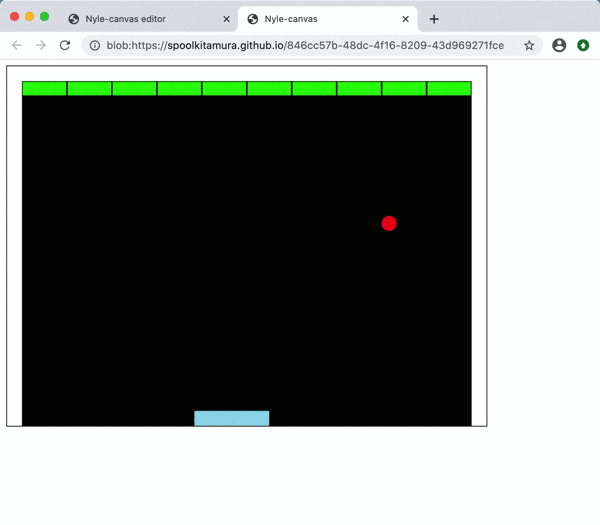### 2-25. Make blocks together (ʻItem class`) (rb_block25.html)

The program for creating / drawing blocks has been repeated 10 times, so I will create them all at once.

Follow the steps below to make it.

#### 1) Create a class called ʻItem class`

When dealing with blocks, it is convenient to be able to handle the following attributes collectively.

--x position --y position --Image --Image width --Image height

Based on that, we will create a class called ʻItem class`.

``````class Item
def initialize(x, y, image)
@x = x
@y = y
@image  = image
@width  = image.width
@height = image.height
end
attr_accessor :x, :y, :image, :width, :height
end
``````

The ʻItem class`(instance) now has a`x position`, a` y position`, a `image`, a` width`, and a `height`.

#### 2) Prepare an image of the block

`img_block = Image.load("block.png ")`

#### 3) Make a block with ʻItem class`

ʻItem.new (x position, y position, img_block) `

#### 4) Prepare the array `blocks` to create multiple blocks (blocks)

Create an empty array `blocks` with`blocks = []`.

#### 5) Create blocks one by one and add them to the array `blocks`

Use the `<<` method to add to the array to create one block and repeat the operation to add it to the array `blocks`.

Since it repeats 10 times, use `10.times do ~ end` to increase` x` each time to make a block with the horizontal position shifted. (`X` changes to 0, 1, 2, ..., 9)

``````10.times do |x|
blocks << Item.new(21 + (img_block.width + 2) * x, 21, img_block)
``````

#### 6) Summarize the drawing of the block group

Bloch group`blocks`Is an array, so repeat the operation`Array.each do |Array要素| 〜 end`Can be used.

``````blocks.each do |block|
Window.draw(block.x, block.y, block.image)
end
``````

With the above, the program is much cleaner. (Comments have also been added.)

#### `rb_block25.rb`

``````
include DX
#Code for initial setting(your setup code here)
Window.width   = 640
Window.height  = 480
Window.bgcolor = C_BLACK

#Wall thickness: left, right, top; 20#◆ Addition (comment)

#Make the block an Item class#◆ Addition (comment)
def initialize(x, y, image)                     #◆ Addition
attr_accessor :x, :y, :image, :width, :height   #◆ Addition

bar_x = 250
bar_y = 480 - img_bar.height
bar_width  = img_bar.width
bar_height = img_bar.height

ball_x = 300
ball_y = 400
dx     =   2
dy     =  -2
ball_width  = img_ball.width
ball_height = img_ball.height

blocks << Item.new(21 + (img_block.width + 2) * x, 21, img_block)   #◆ Addition

#Setting the number of drawings per second (initial value: 60)
#Window.fps = 30

Window.loop do
#bar_x += Input.x * 4       #For left and right keys
bar_x = Input.mouse_x       #For mouse
if bar_x < 20
bar_x = 20
elsif bar_x > 640 - 20 - img_bar.width
bar_x = 640 - 20 - img_bar.width
end

ball_x += dx
ball_y += dy

#If it hits a wall, it bounces back (x direction)#◆ Addition (comment)
if ball_x < 20 || (ball_x + ball_width) > 620
ball_x -= dx
dx = -dx
end

#If it hits the wall, it bounces off(y direction)                       #◆ Addition (comment)
if ball_y < 20
ball_y -= dy
dy = -dy
end

#Collision detection with bar#◆ Addition (comment)
if (ball_y + ball_height) >  (480 - bar_height)  &&
(ball_y + ball_height) <= (480 - bar_height + dy.abs)  &&
(ball_x + ball_width)  >  bar_x               &&
ball_x                 <  (bar_x + bar_width)

ball_y -= dy
dy = -dy
end

Window.draw_box_fill(  0,   0,  20, 480, C_WHITE)
Window.draw_box_fill(620,   0, 640, 480, C_WHITE)
Window.draw_box_fill(  0,   0, 640,  20, C_WHITE)

Window.draw(bar_x, bar_y, img_bar)
Window.draw(ball_x, ball_y, img_ball)

end
``````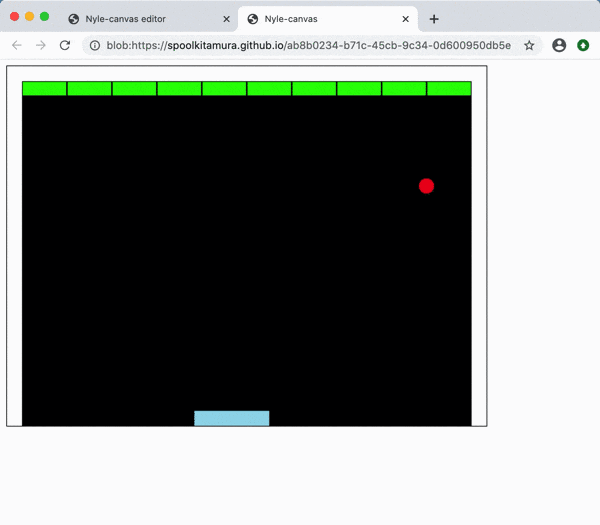### 2-26. Put out a block (make the second stage) (rb_block26.html)

We will also make the second block.

In the second row, the `y position` is increased by`block height (img_block.height) + gap (2)`.

Write using 10.times do ~ end as in the first row.

#### `rb_block26.rb`

``````
include DX
#Code for initial setting(your setup code here)
Window.width   = 640
Window.height  = 480
Window.bgcolor = C_BLACK

#Wall thickness: left, right, top; 20

#Make the block an Item class
class Item
def initialize(x, y, image)
@x = x
@y = y
@image  = image
@width  = image.width
@height = image.height
end
attr_accessor :x, :y, :image, :width, :height
end

#Bar preparation
bar_x = 250
bar_y = 480 - img_bar.height
bar_width  = img_bar.width
bar_height = img_bar.height

#Ball preparation
ball_x = 300
ball_y = 400
dx     =   2
dy     =  -2
ball_width  = img_ball.width
ball_height = img_ball.height

#Block preparation

#Bloch group initialization
blocks = []
10.times do |x|
blocks << Item.new(21 + (img_block.width + 2) * x, 21, img_block)
end

blocks << Item.new(21 + (img_block.width + 2) * x, 21 + (img_block.height + 2), img_block)   #◆ Addition

#Setting the number of drawings per second (initial value: 60)
#Window.fps = 30

#Main loop
Window.loop do
#Move the bar
#bar_x += Input.x * 4       #For left and right keys
bar_x = Input.mouse_x       #For mouse
if bar_x < 20
bar_x = 20
elsif bar_x > 640 - 20 - img_bar.width
bar_x = 640 - 20 - img_bar.width
end

#Move the ball
ball_x += dx
ball_y += dy

#If it hits a wall, it bounces back (x direction)
if ball_x < 20 || (ball_x + ball_width) > 620
ball_x -= dx
dx = -dx
end

#If it hits the wall, it bounces off(y direction)
if ball_y < 20
ball_y -= dy
dy = -dy
end

#Collision detection with bar
if (ball_y + ball_height) >  (480 - bar_height)  &&
(ball_y + ball_height) <= (480 - bar_height + dy.abs)  &&
(ball_x + ball_width)  >  bar_x               &&
ball_x                 <  (bar_x + bar_width)

ball_y -= dy
dy = -dy
end

#Screen drawing
Window.draw_box_fill(  0,   0,  20, 480, C_WHITE)
Window.draw_box_fill(620,   0, 640, 480, C_WHITE)
Window.draw_box_fill(  0,   0, 640,  20, C_WHITE)

Window.draw(bar_x, bar_y, img_bar)
Window.draw(ball_x, ball_y, img_ball)

blocks.each do |block|
Window.draw(block.x, block.y, block.image)
end
end
``````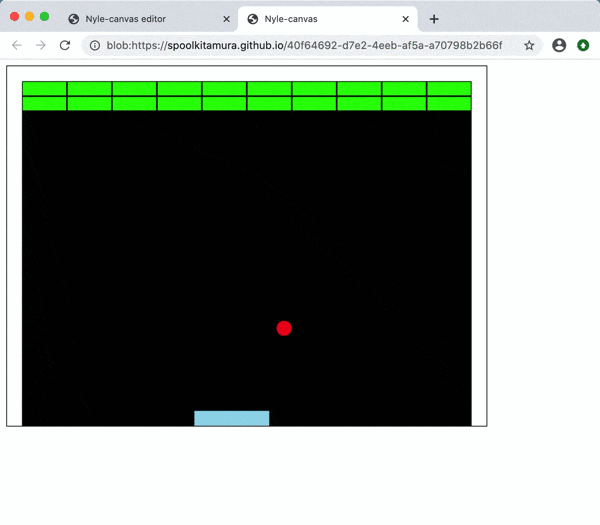### 2-27. Put out a block (make up to the 5th stage) (rb_block27.html)

I made up to the 5th stage.

#### `rb_block27.rb`

``````
include DX
#Code for initial setting(your setup code here)
Window.width   = 640
Window.height  = 480
Window.bgcolor = C_BLACK

#Wall thickness: left, right, top; 20

#Make the block an Item class
class Item
def initialize(x, y, image)
@x = x
@y = y
@image  = image
@width  = image.width
@height = image.height
end
attr_accessor :x, :y, :image, :width, :height
end

#Bar preparation
bar_x = 250
bar_y = 480 - img_bar.height
bar_width  = img_bar.width
bar_height = img_bar.height

#Ball preparation
ball_x = 300
ball_y = 400
dx     =   2
dy     =  -2
ball_width  = img_ball.width
ball_height = img_ball.height

#Block preparation

#Bloch group initialization
blocks = []
10.times do |x|
blocks << Item.new(21 + (img_block.width + 2) * x, 21, img_block)
end

10.times do |x|
blocks << Item.new(21 + (img_block.width + 2) * x, 21 + (img_block.height + 2), img_block)
end

blocks << Item.new(21 + (img_block.width + 2) * x, 21 + (img_block.height + 2) * 2, img_block)   #◆ Addition

blocks << Item.new(21 + (img_block.width + 2) * x, 21 + (img_block.height + 2) * 3, img_block)   #◆ Addition

blocks << Item.new(21 + (img_block.width + 2) * x, 21 + (img_block.height + 2) * 4, img_block)   #◆ Addition

#Setting the number of drawings per second (initial value: 60)
#Window.fps = 30

#Main loop
Window.loop do
#Move the bar
#bar_x += Input.x * 4       #For left and right keys
bar_x = Input.mouse_x       #For mouse
if bar_x < 20
bar_x = 20
elsif bar_x > 640 - 20 - img_bar.width
bar_x = 640 - 20 - img_bar.width
end

#Move the ball
ball_x += dx
ball_y += dy

#If it hits a wall, it bounces back (x direction)
if ball_x < 20 || (ball_x + ball_width) > 620
ball_x -= dx
dx = -dx
end

#If it hits the wall, it bounces off(y direction)
if ball_y < 20
ball_y -= dy
dy = -dy
end

#Collision detection with bar
if (ball_y + ball_height) >  (480 - bar_height)  &&
(ball_y + ball_height) <= (480 - bar_height + dy.abs)  &&
(ball_x + ball_width)  >  bar_x               &&
ball_x                 <  (bar_x + bar_width)

ball_y -= dy
dy = -dy
end

#Screen drawing
Window.draw_box_fill(  0,   0,  20, 480, C_WHITE)
Window.draw_box_fill(620,   0, 640, 480, C_WHITE)
Window.draw_box_fill(  0,   0, 640,  20, C_WHITE)

Window.draw(bar_x, bar_y, img_bar)
Window.draw(ball_x, ball_y, img_ball)

blocks.each do |block|
Window.draw(block.x, block.y, block.image)
end
end
``````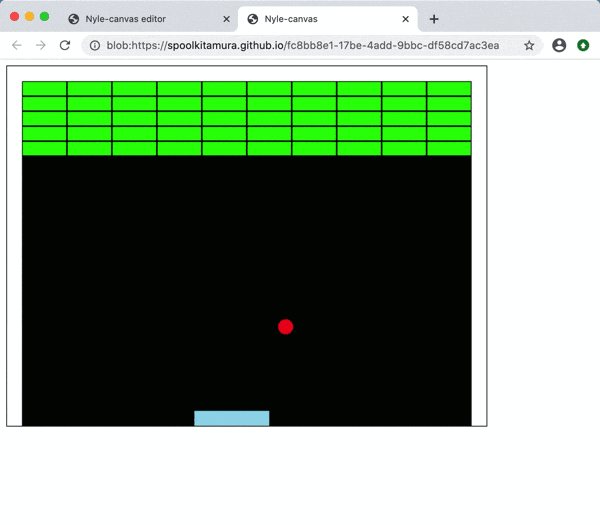### 2-28. Put out blocks (make up to the 5th row) (rb_block28.html)

Since `10.times do ~ end` appeared 5 times, I will summarize this as well.

Put `5.times do ~ end` in` 10.times do ~ end` to make it a double shape.

#### `rb_block28.rb`

``````
include DX
#Code for initial setting(your setup code here)
Window.width   = 640
Window.height  = 480
Window.bgcolor = C_BLACK

#Wall thickness: left, right, top; 20

#Make the block an Item class
class Item
def initialize(x, y, image)
@x = x
@y = y
@image  = image
@width  = image.width
@height = image.height
end
attr_accessor :x, :y, :image, :width, :height
end

#Bar preparation
bar_x = 250
bar_y = 480 - img_bar.height
bar_width  = img_bar.width
bar_height = img_bar.height

#Ball preparation
ball_x = 300
ball_y = 400
dx     =   2
dy     =  -2
ball_width  = img_ball.width
ball_height = img_ball.height

#Block preparation

#Bloch group initialization
blocks = []
10.times do |x|
blocks << Item.new(21 + (img_block.width + 2) * x, 21 + (img_block.height + 2) * y, img_block)  #◆ Addition
end

#Setting the number of drawings per second (initial value: 60)
#Window.fps = 30

#Main loop
Window.loop do
#Move the bar
#bar_x += Input.x * 4       #For left and right keys
bar_x = Input.mouse_x       #For mouse
if bar_x < 20
bar_x = 20
elsif bar_x > 640 - 20 - img_bar.width
bar_x = 640 - 20 - img_bar.width
end

#Move the ball
ball_x += dx
ball_y += dy

#If it hits a wall, it bounces back (x direction)
if ball_x < 20 || (ball_x + ball_width) > 620
ball_x -= dx
dx = -dx
end

#If it hits the wall, it bounces off(y direction)
if ball_y < 20
ball_y -= dy
dy = -dy
end

#Collision detection with bar
if (ball_y + ball_height) >  (480 - bar_height)  &&
(ball_y + ball_height) <= (480 - bar_height + dy.abs)  &&
(ball_x + ball_width)  >  bar_x               &&
ball_x                 <  (bar_x + bar_width)

ball_y -= dy
dy = -dy
end

#Screen drawing
Window.draw_box_fill(  0,   0,  20, 480, C_WHITE)
Window.draw_box_fill(620,   0, 640, 480, C_WHITE)
Window.draw_box_fill(  0,   0, 640,  20, C_WHITE)

Window.draw(bar_x, bar_y, img_bar)
Window.draw(ball_x, ball_y, img_ball)

blocks.each do |block|
Window.draw(block.x, block.y, block.image)
end
end
``````

### 2-29. Balls and bars should also be ʻItem class` (rb_block29.html)

Come to think of it, the attributes of the ball and bar are almost the same as the ʻItem class`, so let's make it the ʻItem class`.

This also makes the program easier to read.

#### `rb_block29.rb`

``````
include DX
#Code for initial setting(your setup code here)
Window.width   = 640
Window.height  = 480
Window.bgcolor = C_BLACK

#Wall thickness: left, right, top; 20

#Balls, bars and blocks should be in the Item class#◇ Change (balls and bars are also remade into Item class)
class Item
def initialize(x, y, image)
@x = x
@y = y
@image  = image
@width  = image.width
@height = image.height
end
attr_accessor :x, :y, :image, :width, :height
end

#Bar preparation
bar = Item.new(250, 480 - img_bar.height, img_bar)  #◇ Change (remake to Item class)

#Ball preparation
ball = Item.new(300, 400, img_ball)                 #◇ Change (remake to Item class)
dx =  2   #Ball speed (x direction)#◇ Change (character alignment, comment addition)
dy = -2   #Ball speed (y direction)#◇ Change (character alignment, comment addition)

#Block preparation

#Bloch group initialization
blocks = []
10.times do |x|
5.times do |y|
blocks << Item.new(21 + (img_block.width + 2) * x, 21 + (img_block.height + 2) * y, img_block)
end
end

#Setting the number of drawings per second (initial value: 60)
#Window.fps = 30

#Main loop
Window.loop do
#Move the bar
#bar.x += Input.x * 4       #For left and right keys#◇ Change (bar.to x)
bar.x = Input.mouse_x       #For mouse#◇ Change (bar.to x)
if bar.x < 20                                #◇ Change (bar.to x)
bar.x = 20                                 #◇ Change (bar.to x)
elsif bar.x > 640 - 20 - bar.width           #◇ Change (bar.x、bar.to width)
bar.x = 640 - 20 - bar.width               #◇ Change (bar.x、bar.to width)
end

#Move the ball
ball.x += dx                                 #◇ Change (ball.to x)
ball.y += dy                                 #◇ Change (ball.to y)

#If it hits a wall, it bounces back (x direction)
if ball.x < 20 || (ball.x + ball.width) > 620  #◇ Change (ball.x、ball.to width)
ball.x -= dx                               #◇ Change (ball.to x)
dx = -dx
end

#If it hits the wall, it bounces off(y direction)
if ball.y < 20                               #◇ Change (ball.to y)
ball.y -= dy                               #◇ Change (ball.to y)
dy = -dy
end

#Collision detection with bar
if (ball.y + ball.height) >  (480 - bar.height)  &&                #◇ Change (ball.y、ball.height、bar.to height)
(ball.y + ball.height) <= (480 - bar.height + dy.abs)  &&       #◇ Change (ball.y、ball.height、bar.to height)
(ball.x + ball.width)  >  bar.x               &&                #◇ Change (ball.x、ball.width、bar.to x)
ball.x                 <  (bar.x + bar.width)                   #◇ Change (ball.x、bar.x、bar.to width)

ball.y -= dy                               #◇ Change (ball.to y)
dy = -dy
end

#Screen drawing
Window.draw_box_fill(  0,   0,  20, 480, C_WHITE)
Window.draw_box_fill(620,   0, 640, 480, C_WHITE)
Window.draw_box_fill(  0,   0, 640,  20, C_WHITE)

Window.draw(bar.x, bar.y, bar.image)        #◇ Change (bar.x、bar.y、bar.To image)
Window.draw(ball.x, ball.y, ball.image)     #◇ Change (ball.x、ball.y、ball.To image)

blocks.each do |block|
Window.draw(block.x, block.y, block.image)
end
end
``````

### 2-30. Create collision detection `collision?` (Rb_block30.html)

In the future, collision detection with blocks as well as bars will be performed many times, so create a collision detection `collision?`. Here, we will consider the rectangles.

Considering the two rectangles ʻitem_a` and ʻitem_b`;

--Coordinates of ʻitem_a`; upper left (ʻa_x0`, ʻa_y0`), lower right (ʻa_x1`, ʻa_y1`)

――Coordinates of ʻitem_b`; upper left (`b_x0`, `b_y0`), lower right (`b_x1`, `b_y1`)

The x-coordinate of ʻitem_a` is ʻa_x0 to a_x1`, and the y-coordinate is ʻa_y0 to a_y1`.

ʻThe x-coordinate of item_b` is` b_x0 to b_x1`, and the y-coordinate is `b_y0 to b_y1`.

Considering each coordinate (x, y), the conditions under which the two collide (collide) are as follows.

``````a_x0 < b_x1 and
a_x1 > b_x0 and

a_y0 < b_y1 and
a_y1 > b_y0
``````

When I code this, it looks like this: (ʻItem_a` and ʻitem_b` are ʻItem class`)

``````def collision?(item_a, item_b)
a_x0 = item_a.x
a_x1 = item_a.x + item_a.width
a_y0 = item_a.y
a_y1 = item_a.y + item_a.height

b_x0 = item_b.x
b_x1 = item_b.x + item_b.width
b_y0 = item_b.y
b_y1 = item_b.y + item_b.height

if a_x0 < b_x1 &&
a_x1 > b_x0 &&
a_y0 < b_y1 &&
a_y1 > b_y0

true
end
end
``````

`collision?` Returns `true` if there is a collision.

(For other collision detection concepts (circles, colors), see below;

→ ・ DXRuby: Let's make "collision detection" by yourself --Qiita https://qiita.com/noanoa07/items/b7d647bba20116c41a77 ）

Also, since it is necessary to consider the direction of bounce (`x direction`,` y direction`), the movement of the ball is divided into the `x direction` and the` y direction`, and collision detection is performed for each.

#### `rb_block30.rb`

``````
include DX
#Code for initial setting(your setup code here)
Window.width   = 640
Window.height  = 480
Window.bgcolor = C_BLACK

#Wall thickness: left, right, top; 20

#Balls, bars and blocks should be in the Item class
class Item
def initialize(x, y, image)
@x = x
@y = y
@image  = image
@width  = image.width
@height = image.height
end
attr_accessor :x, :y, :image, :width, :height
end

a_x1 = item_a.x + item_a.width    #◆ Addition
a_y1 = item_a.y + item_a.height   #◆ Addition
b_x1 = item_b.x + item_b.width    #◆ Addition
b_y1 = item_b.y + item_b.height   #◆ Addition
if a_x0 < b_x1 &&                 #◆ Addition
a_x1 > b_x0 &&                 #◆ Addition
a_y0 < b_y1 &&                 #◆ Addition

#Bar preparation
bar = Item.new(250, 480 - img_bar.height, img_bar)

#Ball preparation
ball = Item.new(300, 400, img_ball)
dx =  2   #Ball speed (x direction)
dy = -2   #Ball speed (y direction)

#Block preparation

#Bloch group initialization
blocks = []
10.times do |x|
5.times do |y|
blocks << Item.new(21 + (img_block.width + 2) * x, 21 + (img_block.height + 2) * y, img_block)
end
end

#Setting the number of drawings per second (initial value: 60)
#Window.fps = 30

#Main loop
Window.loop do
#Move the bar
#bar.x += Input.x * 4       #For left and right keys
bar.x = Input.mouse_x       #For mouse
if bar.x < 20
bar.x = 20
elsif bar.x > 640 - 20 - bar.width
bar.x = 640 - 20 - bar.width
end

#Move the ball in the y direction#◇ Change (only in the y direction)
ball.y += dy                   #◇ Change (only in the y direction)

#Collision detection with bar
if collision?(ball, bar)       #◇ Change (rewrite)
if ball.y + ball.height  <=  480 - bar.height + dy.abs  #◇ Change (rewrite)
ball.y -= dy               #◇ Change (rewrite)
dy = -dy                   #◇ Change (rewrite)
end                          #◇ Change (rewrite)
end                            #◇ Change (rewrite)

#If it hits the wall, it bounces off(y direction)
if ball.y < 20
ball.y -= dy
dy = -dy
end

#Move the ball in the x direction#◇ Change (only in x direction)
ball.x += dx                   #◇ Change (only in x direction)

#If it hits a wall, it bounces back (x direction)
if ball.x < 20 || (ball.x + ball.width) > 620
ball.x -= dx
dx = -dx
end

#Screen drawing
Window.draw_box_fill(  0,   0,  20, 480, C_WHITE)
Window.draw_box_fill(620,   0, 640, 480, C_WHITE)
Window.draw_box_fill(  0,   0, 640,  20, C_WHITE)

Window.draw(bar.x, bar.y, bar.image)
Window.draw(ball.x, ball.y, ball.image)

blocks.each do |block|
Window.draw(block.x, block.y, block.image)
end
end
``````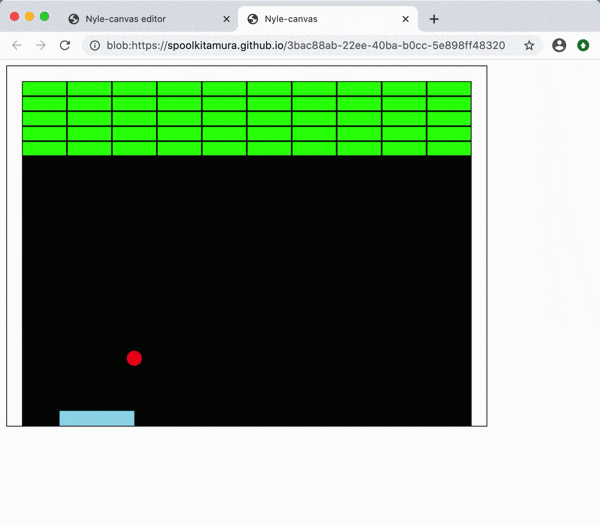### 2-31. Make `collision?` a method of ʻItem class` (rb_block31.html)

In Ruby, the notation ʻitem_a.collision? (Item_b)`is used more often than the notation`collision? (Item_a, item_b)` (object-oriented).

To do this, make `collision?` a method of the ʻItem class` (more precisely, an instance method).

How to make it is as follows.

``````class Item
def Method you want to add
#Method content
end
end
``````

Here, the equivalent of ʻitem_a` is written as` self`.

``````class Item

def collision?(item_b)
a_x0 = self.x
a_x1 = self.x + self.width
a_y0 = self.y
a_y1 = self.y + self.height

b_x0 = item_b.x
b_x1 = item_b.x + item_b.width
b_y0 = item_b.y
b_y1 = item_b.y + item_b.height

if a_x0 < b_x1 &&
a_x1 > b_x0 &&
a_y0 < b_y1 &&
a_y1 > b_y0

true
end
end
end
``````

With this, the conventional way of writing `collision? (Ball, bar)` is changed to `ball.collision? (Bar)`.

#### `rb_block31.rb`

``````
include DX
#Code for initial setting(your setup code here)
Window.width   = 640
Window.height  = 480
Window.bgcolor = C_BLACK

#Wall thickness: left, right, top; 20

#Balls, bars and blocks should be in the Item class
class Item
def initialize(x, y, image)
@x = x
@y = y
@image  = image
@width  = image.width
@height = image.height
end
attr_accessor :x, :y, :image, :width, :height

#Collision detection#◇ Change (to the method of Item class)
def collision?(item_b)              #◇ Change (rewrite)
a_x0 = self.x                     #◇ Change (rewrite)
a_x1 = self.x + self.width        #◇ Change (rewrite)
a_y0 = self.y                     #◇ Change (rewrite)
a_y1 = self.y + self.height       #◇ Change (rewrite)
#◇ Change (rewrite)
b_x0 = item_b.x                   #◇ Change (rewrite)
b_x1 = item_b.x + item_b.width    #◇ Change (rewrite)
b_y0 = item_b.y                   #◇ Change (rewrite)
b_y1 = item_b.y + item_b.height   #◇ Change (rewrite)
#◇ Change (to the method of Item class)
if a_x0 < b_x1 &&                 #◇ Change (to the method of Item class)
a_x1 > b_x0 &&                 #◇ Change (to the method of Item class)
a_y0 < b_y1 &&                 #◇ Change (to the method of Item class)
a_y1 > b_y0                    #◇ Change (to the method of Item class)
#◇ Change (to the method of Item class)
true                            #◇ Change (to the method of Item class)
end                               #◇ Change (to the method of Item class)
end                                 #◇ Change (to the method of Item class)
end                                   #◇ Change (to the method of Item class)

#Bar preparation
bar = Item.new(250, 480 - img_bar.height, img_bar)

#Ball preparation
ball = Item.new(300, 400, img_ball)
dx =  2   #Ball speed (x direction)
dy = -2   #Ball speed (y direction)

#Block preparation

#Bloch group initialization
blocks = []
10.times do |x|
5.times do |y|
blocks << Item.new(21 + (img_block.width + 2) * x, 21 + (img_block.height + 2) * y, img_block)
end
end

#Setting the number of drawings per second (initial value: 60)
#Window.fps = 30

#Main loop
Window.loop do
#Move the bar
#bar.x += Input.x * 4       #For left and right keys
bar.x = Input.mouse_x       #For mouse
if bar.x < 20
bar.x = 20
elsif bar.x > 640 - 20 - bar.width
bar.x = 640 - 20 - bar.width
end

#Move the ball in the y direction
ball.y += dy

#Collision detection with bar
if ball.collision?(bar)        #◇ Change (rewrite)
if ball.y + ball.height  <=  480 - bar.height + dy.abs
ball.y -= dy
dy = -dy
end
end

#If it hits the wall, it bounces off(y direction)
if ball.y < 20
ball.y -= dy
dy = -dy
end

#Move the ball in the x direction
ball.x += dx

#If it hits a wall, it bounces back (x direction)
if ball.x < 20 || (ball.x + ball.width) > 620
ball.x -= dx
dx = -dx
end

#Screen drawing
Window.draw_box_fill(  0,   0,  20, 480, C_WHITE)
Window.draw_box_fill(620,   0, 640, 480, C_WHITE)
Window.draw_box_fill(  0,   0, 640,  20, C_WHITE)

Window.draw(bar.x, bar.y, bar.image)
Window.draw(ball.x, ball.y, ball.image)

blocks.each do |block|
Window.draw(block.x, block.y, block.image)
end
end
``````

### 2-32. Collision detection with block (hit block disappears) (rb_block32.html)

Makes a collision judgment with the block.

`Array.delete_if` is an instruction to retrieve array elements one by one and delete them from the array if the conditions are met.

Therefore, you can delete the blocks that are `true` in the collision detection from the block group array` blocks`.

``````blocks.delete_if do |block|
if ball.collision?(block)
true
end
end
``````

The code using this is as follows.

#### `rb_block32.rb`

``````
include DX
#Code for initial setting(your setup code here)
Window.width   = 640
Window.height  = 480
Window.bgcolor = C_BLACK

#Wall thickness: left, right, top; 20

#Balls, bars and blocks should be in the Item class
class Item
def initialize(x, y, image)
@x = x
@y = y
@image  = image
@width  = image.width
@height = image.height
end
attr_accessor :x, :y, :image, :width, :height

#Collision detection
def collision?(item_b)
a_x0 = self.x
a_x1 = self.x + self.width
a_y0 = self.y
a_y1 = self.y + self.height

b_x0 = item_b.x
b_x1 = item_b.x + item_b.width
b_y0 = item_b.y
b_y1 = item_b.y + item_b.height

if a_x0 < b_x1 &&
a_x1 > b_x0 &&
a_y0 < b_y1 &&
a_y1 > b_y0

true
end
end
end

#Bar preparation
bar = Item.new(250, 480 - img_bar.height, img_bar)

#Ball preparation
ball = Item.new(300, 400, img_ball)
dx =  2   #Ball speed (x direction)
dy = -2   #Ball speed (y direction)

#Block preparation

#Bloch group initialization
blocks = []
10.times do |x|
5.times do |y|
blocks << Item.new(21 + (img_block.width + 2) * x, 21 + (img_block.height + 2) * y, img_block)
end
end

#Setting the number of drawings per second (initial value: 60)
#Window.fps = 30

#Main loop
Window.loop do
#Move the bar
#bar.x += Input.x * 4       #For left and right keys
bar.x = Input.mouse_x       #For mouse
if bar.x < 20
bar.x = 20
elsif bar.x > 640 - 20 - bar.width
bar.x = 640 - 20 - bar.width
end

#Move the ball in the y direction
ball.y += dy

#Collision detection with bar
if ball.collision?(bar)
if ball.y + ball.height  <=  480 - bar.height + dy.abs
ball.y -= dy
dy = -dy
end
end

#Collision detection with block (y direction)#◆ Addition

#If it hits the wall, it bounces off(y direction)
if ball.y < 20
ball.y -= dy
dy = -dy
end

#Move the ball in the x direction
ball.x += dx

#Collision detection with block (x direction)#◆ Addition

#If it hits a wall, it bounces back (x direction)
if ball.x < 20 || (ball.x + ball.width) > 620
ball.x -= dx
dx = -dx
end

#Screen drawing
Window.draw_box_fill(  0,   0,  20, 480, C_WHITE)
Window.draw_box_fill(620,   0, 640, 480, C_WHITE)
Window.draw_box_fill(  0,   0, 640,  20, C_WHITE)

Window.draw(bar.x, bar.y, bar.image)
Window.draw(ball.x, ball.y, ball.image)

blocks.each do |block|
Window.draw(block.x, block.y, block.image)
end
end
``````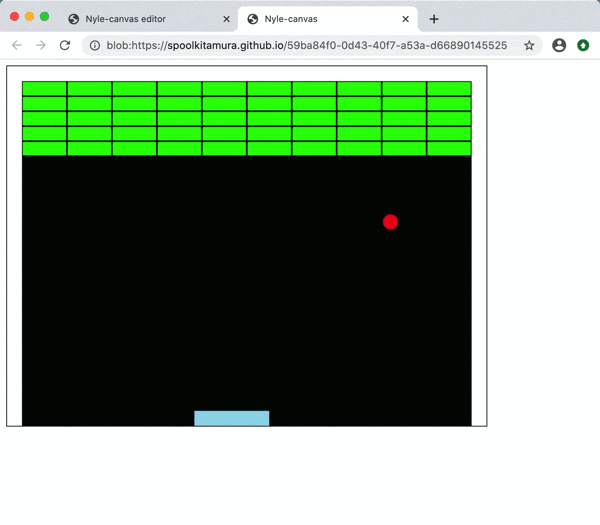### 2-33. If it hits a block, it bounces off; it's completed (rb_block33.html)

When it hits the block, it will bounce off.

If it hits a block, just add the bouncing code.

``````#Collision detection with block (y direction)
blocks.delete_if do |block|
if ball.collision?(block)
true
end
end
``````

This completes "Breakout"!

#### `rb_block33.rb`

``````
include DX
#Code for initial setting(your setup code here)
Window.width   = 640
Window.height  = 480
Window.bgcolor = C_BLACK

#Wall thickness: left, right, top; 20

#Balls, bars and blocks should be in the Item class
class Item
def initialize(x, y, image)
@x = x
@y = y
@image  = image
@width  = image.width
@height = image.height
end
attr_accessor :x, :y, :image, :width, :height

#Collision detection
def collision?(item_b)
a_x0 = self.x
a_x1 = self.x + self.width
a_y0 = self.y
a_y1 = self.y + self.height

b_x0 = item_b.x
b_x1 = item_b.x + item_b.width
b_y0 = item_b.y
b_y1 = item_b.y + item_b.height

if a_x0 < b_x1 &&
a_x1 > b_x0 &&
a_y0 < b_y1 &&
a_y1 > b_y0

true
end
end
end

#Bar preparation
bar = Item.new(250, 480 - img_bar.height, img_bar)

#Ball preparation
ball = Item.new(300, 400, img_ball)
dx =  2   #Ball speed (x direction)
dy = -2   #Ball speed (y direction)

#Block preparation

#Bloch group initialization
blocks = []
10.times do |x|
5.times do |y|
blocks << Item.new(21 + (img_block.width + 2) * x, 21 + (img_block.height + 2) * y, img_block)
end
end

#Setting the number of drawings per second (initial value: 60)
#Window.fps = 30

#Main loop
Window.loop do
#Move the bar
#bar.x += Input.x * 4       #For left and right keys
bar.x = Input.mouse_x       #For mouse
if bar.x < 20
bar.x = 20
elsif bar.x > 640 - 20 - bar.width
bar.x = 640 - 20 - bar.width
end

#Move the ball in the y direction
ball.y += dy

#Collision detection with bar
if ball.collision?(bar)
if ball.y + ball.height  <=  480 - bar.height + dy.abs
ball.y -= dy
dy = -dy
end
end

#Collision detection with block (y direction)
blocks.delete_if do |block|
if ball.collision?(block)
true
end
end

#If it hits the wall, it bounces off(y direction)
if ball.y < 20
ball.y -= dy
dy = -dy
end

#Move the ball in the x direction
ball.x += dx

#Collision detection with block (x direction)
blocks.delete_if do |block|
if ball.collision?(block)
true
end
end

#If it hits a wall, it bounces back (x direction)
if ball.x < 20 || (ball.x + ball.width) > 620
ball.x -= dx
dx = -dx
end

#Screen drawing
Window.draw_box_fill(  0,   0,  20, 480, C_WHITE)
Window.draw_box_fill(620,   0, 640, 480, C_WHITE)
Window.draw_box_fill(  0,   0, 640,  20, C_WHITE)

Window.draw(bar.x, bar.y, bar.image)
Window.draw(ball.x, ball.y, ball.image)

blocks.each do |block|
Window.draw(block.x, block.y, block.image)
end
end
``````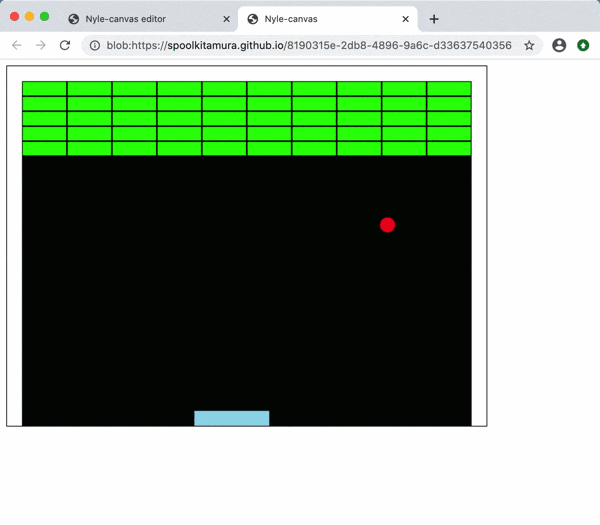# Application problem

## Let's improve and develop "Breakout"

Let's improve and develop "Breakout".

The following is just one example. Feel free to develop it yourself.

## A. Improve operation

Let's try moving the "breakout" that you made and fix the part that you are worried about.

## B. Extend functionality

Let's add the "breakout" function and develop it.

### B-1. Display characters on the screen (rb_block34.html)

Let's display the characters on the screen.

First, prepare the font by default.

`font = Font.new(24)`

The characters are displayed as follows.

`Window.draw_font (x position, y position, string, font, {: color => text color)`

To find out the number of remaining blocks, check the number of elements in the block array `blocks`, so use` blocks.size`.

#### `rb_block34.rb`

``````
include DX
#Code for initial setting(your setup code here)
Window.width   = 640
Window.height  = 480
Window.bgcolor = C_BLACK

#Wall thickness: left, right, top; 20

#Balls, bars and blocks should be in the Item class
class Item
def initialize(x, y, image)
@x = x
@y = y
@image  = image
@width  = image.width
@height = image.height
end
attr_accessor :x, :y, :image, :width, :height

#Collision detection
def collision?(item_b)
a_x0 = self.x
a_x1 = self.x + self.width
a_y0 = self.y
a_y1 = self.y + self.height

b_x0 = item_b.x
b_x1 = item_b.x + item_b.width
b_y0 = item_b.y
b_y1 = item_b.y + item_b.height

if a_x0 < b_x1 &&
a_x1 > b_x0 &&
a_y0 < b_y1 &&
a_y1 > b_y0

true
end
end
end

#Bar preparation
bar = Item.new(250, 480 - img_bar.height, img_bar)

#Ball preparation
ball = Item.new(300, 400, img_ball)
dx =  2   #Ball speed (x direction)
dy = -2   #Ball speed (y direction)

#Block preparation

#Bloch group initialization
blocks = []
10.times do |x|
5.times do |y|
blocks << Item.new(21 + (img_block.width + 2) * x, 21 + (img_block.height + 2) * y, img_block)
end
end

#Setting the number of drawings per second (initial value: 60)
#Window.fps = 30

#Main loop
Window.loop do
#Move the bar
#bar.x += Input.x * 4       #For left and right keys
bar.x = Input.mouse_x       #For mouse
if bar.x < 20
bar.x = 20
elsif bar.x > 640 - 20 - bar.width
bar.x = 640 - 20 - bar.width
end

#Move the ball in the y direction
ball.y += dy

#Collision detection with bar
if ball.collision?(bar)
if ball.y + ball.height  <=  480 - bar.height + dy.abs
ball.y -= dy
dy = -dy
end
end

#Collision detection with block (y direction)
blocks.delete_if do |block|
if ball.collision?(block)
ball.y -= dy
dy = -dy
true
end
end

#If it hits the wall, it bounces off(y direction)
if ball.y < 20
ball.y -= dy
dy = -dy
end

#Move the ball in the x direction
ball.x += dx

#Collision detection with block (x direction)
blocks.delete_if do |block|
if ball.collision?(block)
ball.x -= dx
dx = -dx
true
end
end

#If it hits a wall, it bounces back (x direction)
if ball.x < 20 || (ball.x + ball.width) > 620
ball.x -= dx
dx = -dx
end

#Screen drawing
Window.draw_box_fill(  0,   0,  20, 480, C_WHITE)
Window.draw_box_fill(620,   0, 640, 480, C_WHITE)
Window.draw_box_fill(  0,   0, 640,  20, C_WHITE)

Window.draw(bar.x, bar.y, bar.image)
Window.draw(ball.x, ball.y, ball.image)

blocks.each do |block|
Window.draw(block.x, block.y, block.image)
end

string = "The remaining blocks#{blocks.size}It is an individual."                      #◆ Addition
Window.draw_font(20, 200, string, font, {:color => C_YELLOW})     #◆ Addition
end
``````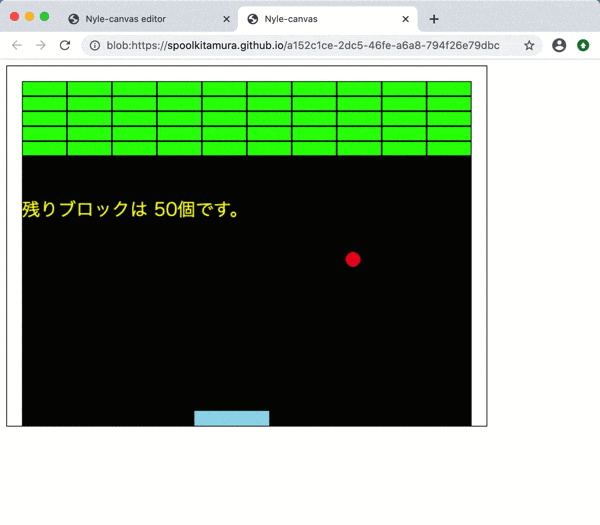### B-2. Add game over screen (rb_block35.html)

Let's add a game over screen.

When the `y position` (` ball.y`) of the ball is larger than the vertical width of the window `480`, the" game over screen "is displayed.

The game over screen is made as follows.

1. Draw a white rectangle the same size as the window size (640, 480) (`Window.draw_box_fill (0, 0, 640, 480, C_WHITE)`)

2. Display the word "Game Over" (`Window.draw_font (200, 200," Game Over ", font, {: color => C_BLACK})`)

#### `rb_block35.rb`

``````
include DX
#Code for initial setting(your setup code here)
Window.width   = 640
Window.height  = 480
Window.bgcolor = C_BLACK

#Wall thickness: left, right, top; 20

#Balls, bars and blocks should be in the Item class
class Item
def initialize(x, y, image)
@x = x
@y = y
@image  = image
@width  = image.width
@height = image.height
end
attr_accessor :x, :y, :image, :width, :height

#Collision detection
def collision?(item_b)
a_x0 = self.x
a_x1 = self.x + self.width
a_y0 = self.y
a_y1 = self.y + self.height

b_x0 = item_b.x
b_x1 = item_b.x + item_b.width
b_y0 = item_b.y
b_y1 = item_b.y + item_b.height

if a_x0 < b_x1 &&
a_x1 > b_x0 &&
a_y0 < b_y1 &&
a_y1 > b_y0

true
end
end
end

#Bar preparation
bar = Item.new(250, 480 - img_bar.height, img_bar)

#Ball preparation
ball = Item.new(300, 400, img_ball)
dx =  2   #Ball speed (x direction)
dy = -2   #Ball speed (y direction)

#Block preparation

#Bloch group initialization
blocks = []
10.times do |x|
5.times do |y|
blocks << Item.new(21 + (img_block.width + 2) * x, 21 + (img_block.height + 2) * y, img_block)
end
end

#Font preparation
font = Font.new(24)

#Setting the number of drawings per second (initial value: 60)
#Window.fps = 30

#Main loop
Window.loop do
#Move the bar
#bar.x += Input.x * 4       #For left and right keys
bar.x = Input.mouse_x       #For mouse
if bar.x < 20
bar.x = 20
elsif bar.x > 640 - 20 - bar.width
bar.x = 640 - 20 - bar.width
end

#Move the ball in the y direction
ball.y += dy

#Collision detection with bar
if ball.collision?(bar)
if ball.y + ball.height  <=  480 - bar.height + dy.abs
ball.y -= dy
dy = -dy
end
end

#Collision detection with block (y direction)
blocks.delete_if do |block|
if ball.collision?(block)
ball.y -= dy
dy = -dy
true
end
end

#If it hits the wall, it bounces off(y direction)
if ball.y < 20
ball.y -= dy
dy = -dy
end

#Move the ball in the x direction
ball.x += dx

#Collision detection with block (x direction)
blocks.delete_if do |block|
if ball.collision?(block)
ball.x -= dx
dx = -dx
true
end
end

#If it hits a wall, it bounces back (x direction)
if ball.x < 20 || (ball.x + ball.width) > 620
ball.x -= dx
dx = -dx
end

#Screen drawing
Window.draw_box_fill(  0,   0,  20, 480, C_WHITE)
Window.draw_box_fill(620,   0, 640, 480, C_WHITE)
Window.draw_box_fill(  0,   0, 640,  20, C_WHITE)

Window.draw(bar.x, bar.y, bar.image)
Window.draw(ball.x, ball.y, ball.image)

blocks.each do |block|
Window.draw(block.x, block.y, block.image)
end

#Character display
string = "The remaining blocks#{blocks.size}It is an individual."
Window.draw_font(20, 200, string, font, {:color => C_YELLOW})

if ball.y >= 480                                                          #◆ Addition
Window.draw_box_fill(0, 0, 640, 480, C_WHITE)                           #◆ Addition
Window.draw_font(200, 200, "Game over", font, {:color => C_BLACK})   #◆ Addition

end
``````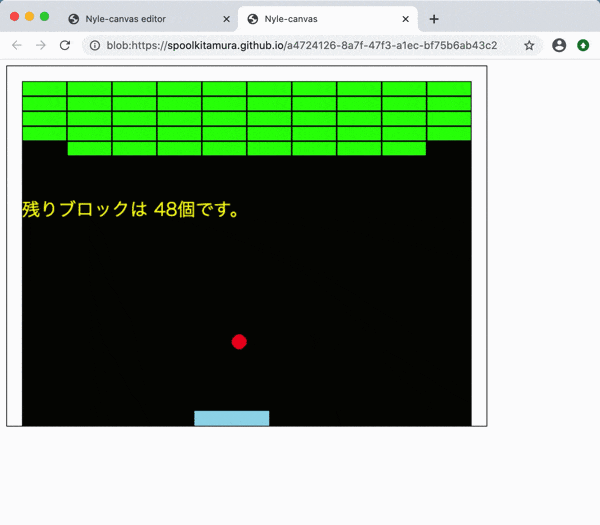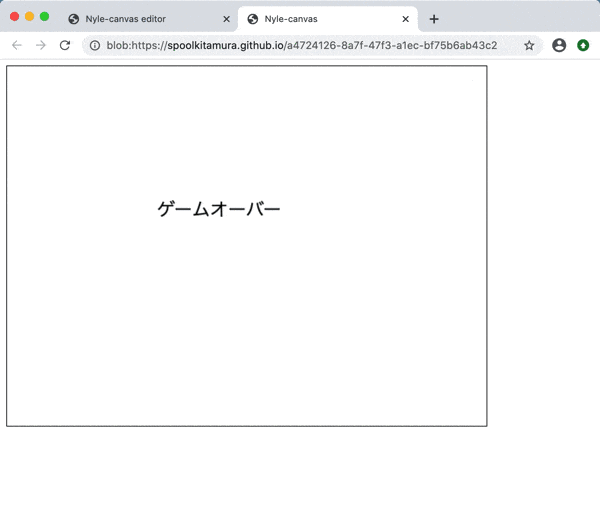### B-3. Restart from the game over screen (rb_block36.html)

From the game over screen, press a specific key to restart the game.

Check ʻInput.key_down? (Keyboard constant)` to see if a key has been pressed.

After that, you are free to set the restart screen. (Here, the remaining blocks are left as they are, and the ball is returned to the initial conditions.)

#### `rb_block36.rb`

``````
include DX
#Code for initial setting(your setup code here)
Window.width   = 640
Window.height  = 480
Window.bgcolor = C_BLACK

#Wall thickness: left, right, top; 20

#Balls, bars and blocks should be in the Item class
class Item
def initialize(x, y, image)
@x = x
@y = y
@image  = image
@width  = image.width
@height = image.height
end
attr_accessor :x, :y, :image, :width, :height

#Collision detection
def collision?(item_b)
a_x0 = self.x
a_x1 = self.x + self.width
a_y0 = self.y
a_y1 = self.y + self.height

b_x0 = item_b.x
b_x1 = item_b.x + item_b.width
b_y0 = item_b.y
b_y1 = item_b.y + item_b.height

if a_x0 < b_x1 &&
a_x1 > b_x0 &&
a_y0 < b_y1 &&
a_y1 > b_y0

true
end
end
end

#Bar preparation
bar = Item.new(250, 480 - img_bar.height, img_bar)

#Ball preparation
ball = Item.new(300, 400, img_ball)
dx =  2   #Ball speed (x direction)
dy = -2   #Ball speed (y direction)

#Block preparation

#Bloch group initialization
blocks = []
10.times do |x|
5.times do |y|
blocks << Item.new(21 + (img_block.width + 2) * x, 21 + (img_block.height + 2) * y, img_block)
end
end

#Font preparation
font = Font.new(24)

#Setting the number of drawings per second (initial value: 60)
#Window.fps = 30

#Main loop
Window.loop do
#Move the bar
#bar.x += Input.x * 4       #For left and right keys
bar.x = Input.mouse_x       #For mouse
if bar.x < 20
bar.x = 20
elsif bar.x > 640 - 20 - bar.width
bar.x = 640 - 20 - bar.width
end

#Move the ball in the y direction
ball.y += dy

#Collision detection with bar
if ball.collision?(bar)
if ball.y + ball.height  <=  480 - bar.height + dy.abs
ball.y -= dy
dy = -dy
end
end

#Collision detection with block (y direction)
blocks.delete_if do |block|
if ball.collision?(block)
ball.y -= dy
dy = -dy
true
end
end

#If it hits the wall, it bounces off(y direction)
if ball.y < 20
ball.y -= dy
dy = -dy
end

#Move the ball in the x direction
ball.x += dx

#Collision detection with block (x direction)
blocks.delete_if do |block|
if ball.collision?(block)
ball.x -= dx
dx = -dx
true
end
end

#If it hits a wall, it bounces back (x direction)
if ball.x < 20 || (ball.x + ball.width) > 620
ball.x -= dx
dx = -dx
end

#Screen drawing
Window.draw_box_fill(  0,   0,  20, 480, C_WHITE)
Window.draw_box_fill(620,   0, 640, 480, C_WHITE)
Window.draw_box_fill(  0,   0, 640,  20, C_WHITE)

Window.draw(bar.x, bar.y, bar.image)
Window.draw(ball.x, ball.y, ball.image)

blocks.each do |block|
Window.draw(block.x, block.y, block.image)
end

#Character display
string = "The remaining blocks#{blocks.size}It is an individual."
Window.draw_font(20, 200, string, font, {:color => C_YELLOW})

#Game over screen
if ball.y >= 480
Window.draw_box_fill(0, 0, 640, 480, C_WHITE)
Window.draw_font(200, 200, "Game over", font, {:color => C_BLACK})
Window.draw_font(200, 230, "Continue with spacebar", font, {:color => C_BLACK})  #◆ Addition